# 1 3 way factorial design. A completely randomized, “between subjects” factorial design

The challenge of analyzing a 3 way factorial design is not in the specification and analysis of the omnibus model (`aov`, `lm`, afex`,`granova`), it is in the follow up analyses.

With the use of the phia and emmeans packages we can obtain Simple 2-way interactions, Simple Simple Main Effects, Simple Main Effects, and orthogonally partitioned contrasts (and non-orthogonal ones) on all sources of variance, e.g., 3-, and 2-way interaction contrasts, main effect contrasts, simple main effect contrasts.

We still need:

1. easier ways to compute effect sizes, especially for contrasts

2. bayesian and robust methods

In order to use this tutorial effectively, the reader should be alert to terminology specific to the style of ANOVA analysis that is traditional in the textbooks such as Kirk, Winer, Keppel, and Maxwell/Delaney/Kelley. The relevant terminology/notation includes:

An AxBxC design: Three independent variables, perhaps called A, B, and C. Fully “between groups” design where participants/cases are measured only once on the outcome variable under the combined conditions of factors A, B, and C.

Levels: The groups defined by each variable are called levels. E.g., in the primary example used in this document the “Grade” factor (factor C) has two “levels”: fifth grade and twelfth grade.

Factorial: The design is called a factorial since each level of each factor is found in all possible combinations with all levels of all other factors. So, for example a 3x3x2 factorial design (AxBxC) has 18 total combinations or “cells”

Cell means vs Marginal Means, Omnibus Effects: Cells are defined by the three way combination of factors. The 3x3x2 design used in this document has 18 cells. But we can imaging “collapsing” on one or more IVs to produce marginal sets of means. For example collapsing on factor C produces an AxB matrix of means (9 means) and that would lead to assessment of the omnibus AxB interaction term. The omnibus terms in a 3 way factorial are the three main effects, the three two way interactions and the three way interaction.

Simple Effects When effects of one or more factors are examined at only specific levels of one or more other factor, then those effects are “simple” These can include:

Simple Main effects: effects of one IV at levels of another factor, but collapsed on the third. E.g., A at B1 or C at A3. These are 1-way questions about the effect of a single IV but the location of the questions is specified by a level of a second variable.

Simple Simple Main effects: The same 1way idea can be extended to effects of one factor at combined levels of two other factors. For example - B at A3,C2

Simple interactions: It is possible to ask a two way interaction question (e.g., AxB), not in an omnibus sense, but at a level of a third IV. For example - AxB at C2

An important part of the grasp of the logic is the understanding of these following concepts:

1. A three way interaction is a test of whether simple 2 way interactions differ across levels of a 3rd variable, e.g., AxB at C1 is not the same as AxB at C2
2. A simple 2 way interaction is a test of whether simple simple main effects differ.
3. An omnibus 2 way interaction tests whether simple main effects differ.
4. A 3 way interaction contrast tests whether simple 2way interaction contrasts differ across levels of a third factor.
5. 2 way interaction contrasts test whether simple main effect contrasts differ.

In addition, many sections of this tutorial rely on the idea of creating orthogonal sets of contrasts. This philosophy derives from the alignment of that approach with theory-drive a priori hypotheses and from the recognized value of full rank models in ANovA work.

# 2 First, load packages that are used by this document

``````library(psych,quietly=TRUE, warn.conflicts=FALSE)
library(afex,quietly=TRUE, warn.conflicts=FALSE)
library(car,quietly=TRUE, warn.conflicts=FALSE)
library(phia,quietly=TRUE, warn.conflicts=FALSE)
library(nortest,quietly=TRUE, warn.conflicts=FALSE)
library(lmtest,quietly=TRUE, warn.conflicts=FALSE)
library(sciplot,quietly=TRUE, warn.conflicts=FALSE)
library(ggplot2,quietly=TRUE, warn.conflicts=FALSE)
library(ggthemes,quietly=TRUE, warn.conflicts=FALSE)
library(plyr,quietly=TRUE, warn.conflicts=FALSE)
library(Rmisc,quietly=TRUE, warn.conflicts=FALSE)
library(knitr,quietly=TRUE, warn.conflicts=FALSE)
library(gt,quietly=TRUE, warn.conflicts=FALSE)
library(emmeans,quietly=TRUE, warn.conflicts=FALSE)
library(tibble,quietly=TRUE, warn.conflicts=FALSE)``````

## 2.1 Read the data from a .csv file

The data are from Keppel and Wickens, pg 466 data set, a 3x3x2 design it is the “keppel_3way_pg466.csv” file. The dependent variable is number of words recalled from a memorized list. Three independent variables were involved and factorially arranged. Participants were either fifth or twelfth graders. They were assigned to one of three Feedback conditions: control (none), praise, or negative. The words were one of three types: “LF_LE”, “HF_LE”, or “HF_HE”. The factorial arrangement of these three factors thus produced a total of eighteen cells in the design.

``````bg.3way <- read.csv("keppel_3way_pg466.csv",header=T, stringsAsFactors=TRUE)
# examine the contents of a few lines and the structure of the data frame
1 none LF_LE fifth 7
2 none LF_LE fifth 7
3 none LF_LE fifth 9
4 none LF_LE fifth 10
5 none LF_LE fifth 9
6 none HF_LE fifth 7
``str(bg.3way)``
``````## 'data.frame':    90 obs. of  5 variables:
##  \$ snum     : int  1 2 3 4 5 6 7 8 9 10 ...
##  \$ feedback : Factor w/ 3 levels "neg","none","pos": 2 2 2 2 2 2 2 2 2 2 ...
##  \$ wordtype : Factor w/ 3 levels "HF_HE","HF_LE",..: 3 3 3 3 3 2 2 2 2 2 ...
##  \$ grade    : Factor w/ 2 levels "fifth","twelfth": 1 1 1 1 1 1 1 1 1 1 ...
##  \$ numrecall: int  7 7 9 10 9 7 8 9 10 10 ...``````

## 2.2 Rework some data defninition characteristics

Some housekeeping with data definition helps format output.

``````# change values of feedback variable to whole words to facilitate
# recode function in car is useful
# library(car)
bg.3way\$feedback <- car::recode(bg.3way\$feedback, "'none'='None'; 'pos'='Positive'; 'neg'='Negative'")``````
``````# define the order of factor labels so that they don't just
# get reported and graphed in alphabetical order
bg.3way\$feedback <- factor(bg.3way\$feedback,
levels=c("None","Positive","Negative"))
bg.3way\$wordtype <- factor(bg.3way\$wordtype,
levels=c("LF_LE","HF_LE","HF_HE"))                  ``````
``````# convert the snum variable to a factor for better
# compatibility with afex functions
bg.3way\$snum <- as.factor(bg.3way\$snum)``````

In order to make writing code easier for the graphing functions, the data frame is attached here. Prior to the ANOVA work it will be detached.

``attach(bg.3way)``
``````# look at contents of the final data frame
1 1 None LF_LE fifth 7
2 2 None LF_LE fifth 7
3 3 None LF_LE fifth 9
4 4 None LF_LE fifth 10
5 5 None LF_LE fifth 9
6 6 None HF_LE fifth 7
7 7 None HF_LE fifth 8
8 8 None HF_LE fifth 9
9 9 None HF_LE fifth 10
NA NA NA NA
87 87 Negative HF_HE twelfth 5
88 88 Negative HF_HE twelfth 7
89 89 Negative HF_HE twelfth 8
90 90 Negative HF_HE twelfth 9

## 2.3 Exploratory Data Analysis

Numeric and graphical summaries are obtained here.

### 2.3.1 Numerical summaries of the dependent variable, by group.

First, use the psych package to look at descriptives.

``````# library(psych)
item group1 group2 vars n mean sd median trimmed mad min max range skew kurtosis se
X11 1 None fifth 1 15 8.400000 1.298351 9 8.384615 1.4826 7 10 3 0.0271129 -1.8430822 0.3352327
X12 2 Positive fifth 1 15 6.733333 2.086236 7 6.846154 2.9652 3 9 6 -0.5715517 -0.7482937 0.5386638
X13 3 Negative fifth 1 15 6.466667 2.325838 7 6.538462 2.9652 2 10 8 -0.4228487 -0.6004418 0.6005289
X14 4 None twelfth 1 15 8.333333 1.290994 8 8.307692 1.4826 6 11 5 0.1957772 0.1252747 0.3333333
X15 5 Positive twelfth 1 15 7.866667 1.457330 8 7.846154 1.4826 6 10 4 -0.0551506 -1.3885181 0.3762809
X16 6 Negative twelfth 1 15 7.800000 1.424279 8 7.846154 1.4826 5 10 5 -0.4518451 -0.6318191 0.3677473

## 2.4 Draw a few graphs to examine the cell means.

Graphical exploration of several types

### 2.4.1 First, a clustered box plot

Use the standard `boxplot` function from base R. Notice how the labels are not always fully visible. It is possible to use other functions to angle the labels so they can be seen - not worked out here.

``````# win.graph()
main="3-way Factorial Design Example",
xlab="Treatment Group",
ylab="NumRecall")``````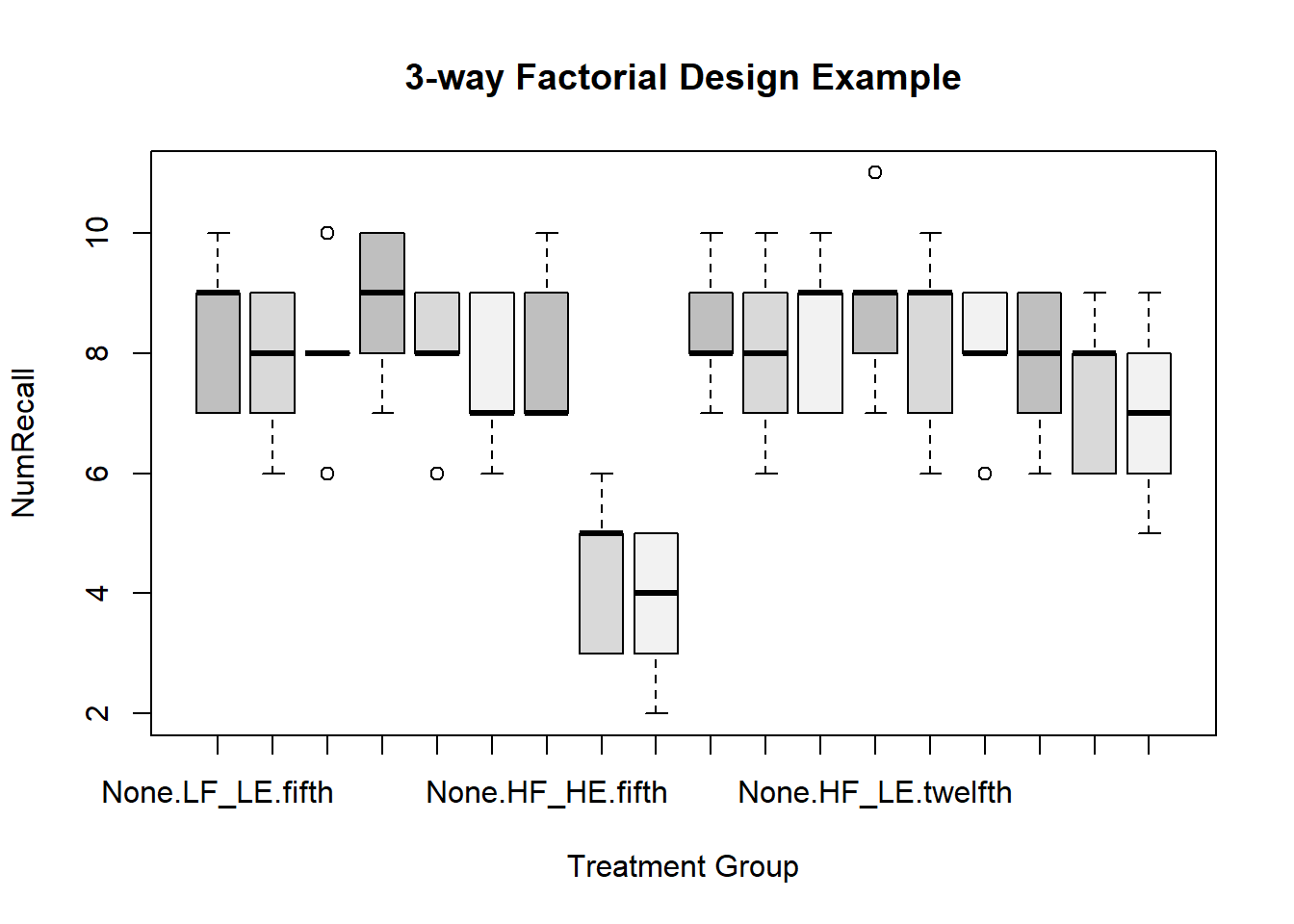A different approach to clustered boxplots can be implemented with ggplot2.

``````# first, summarize the data set to produce a new data frame that ggplot can use
myData <- Rmisc::summarySE(data=bg.3way,measurevar="numrecall", groupvars=c("feedback", "wordtype", "grade"))
# look at the new data frame that contains the summary statistics
myData``````
feedback wordtype grade N numrecall sd se ci
None LF_LE fifth 5 8.4 1.341641 0.6000000 1.665867
None LF_LE twelfth 5 8.4 1.140175 0.5099020 1.415715
None HF_LE fifth 5 8.8 1.303840 0.5830952 1.618932
None HF_LE twelfth 5 8.8 1.483240 0.6633250 1.841685
None HF_HE fifth 5 8.0 1.414214 0.6324555 1.755978
None HF_HE twelfth 5 7.8 1.303840 0.5830952 1.618932
Positive LF_LE fifth 5 7.8 1.303840 0.5830952 1.618932
Positive LF_LE twelfth 5 8.0 1.581139 0.7071068 1.963243
Positive HF_LE fifth 5 8.0 1.224745 0.5477226 1.520722
Positive HF_LE twelfth 5 8.2 1.643168 0.7348469 2.040262
Positive HF_HE fifth 5 4.4 1.341641 0.6000000 1.665867
Positive HF_HE twelfth 5 7.4 1.341641 0.6000000 1.665867
Negative LF_LE fifth 5 8.0 1.414214 0.6324555 1.755978
Negative LF_LE twelfth 5 8.4 1.341641 0.6000000 1.665867
Negative HF_LE fifth 5 7.6 1.341641 0.6000000 1.665867
Negative HF_LE twelfth 5 8.0 1.224745 0.5477226 1.520722
Negative HF_HE fifth 5 3.8 1.303840 0.5830952 1.618932
Negative HF_HE twelfth 5 7.0 1.581139 0.7071068 1.963243
``````p <- ggplot(data=bg.3way, aes(x=feedback, y=numrecall, fill=wordtype)) +
geom_boxplot() +
scale_fill_manual(values = c("grey45", "grey65", "grey75")) +

p``````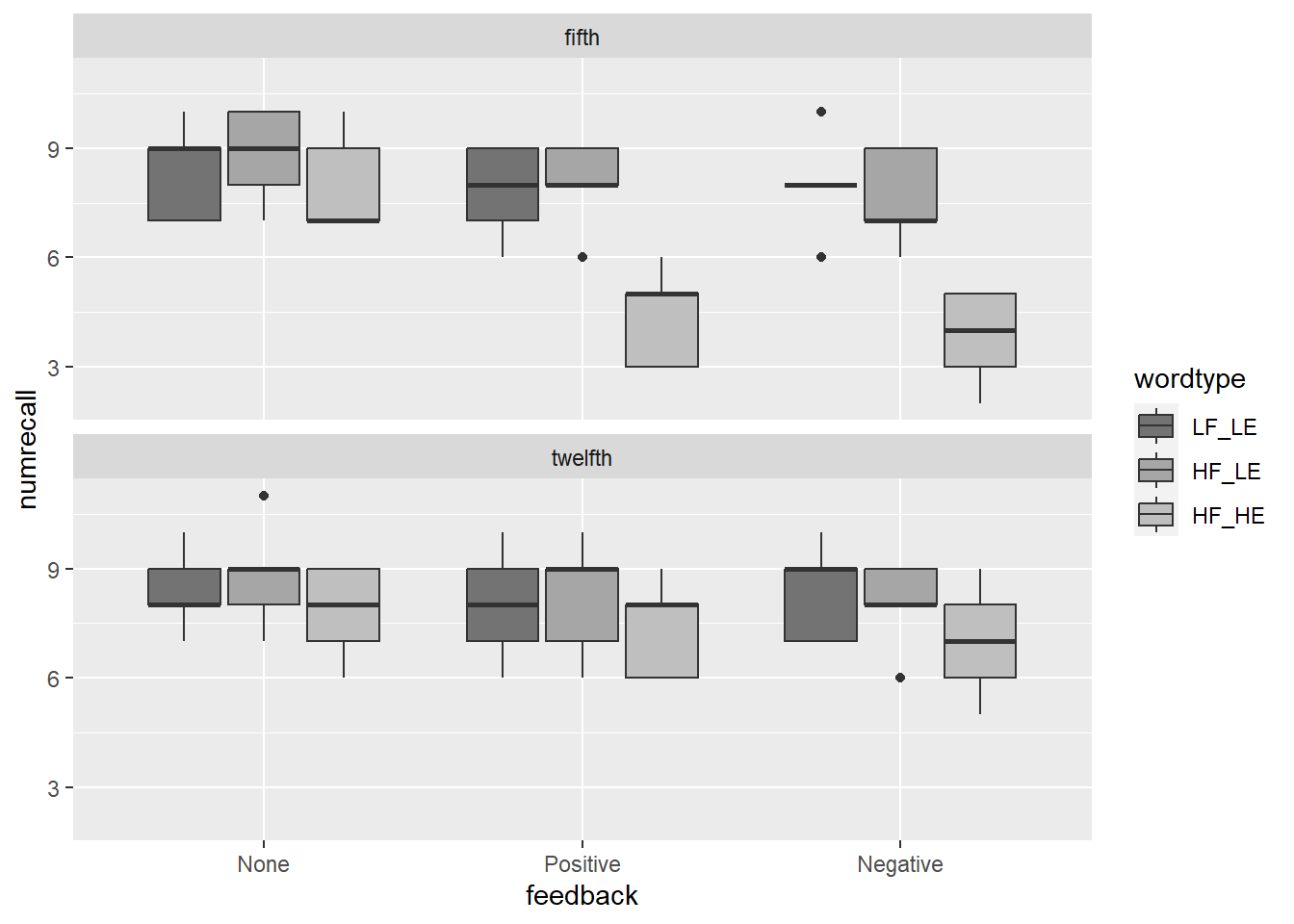### 2.4.2 Bar graphs +/- std error bars

Several approaches to the standard bar graph are available. First, use the `bargraph.CI` function from the sciplot package. The key to producing side by side panels is to use the `screen` function.

``````# Now, bar graphs +/- std error bars
# library(sciplot)
# win.graph()
split.screen(figs=c(1,2))``````
``##  1 2``
``````#win.graph()
screen(1)
bargraph.CI(wordtype,numrecall,group=feedback,lc=TRUE, uc=TRUE,legend=T,
cex.leg=1,bty="n",col=c("gray65","gray80","gray95"),ylim=c(0.01,12),
box()
axis(4,labels=F)
screen(2)
#win.graph()
bargraph.CI(wordtype,numrecall,group=feedback,lc=TRUE, uc=TRUE,legend=T,
cex.leg=1,bty="n",col=c("gray65","gray80","gray95"),ylim=c(0.01,12),
box()
axis(4,labels=F)``````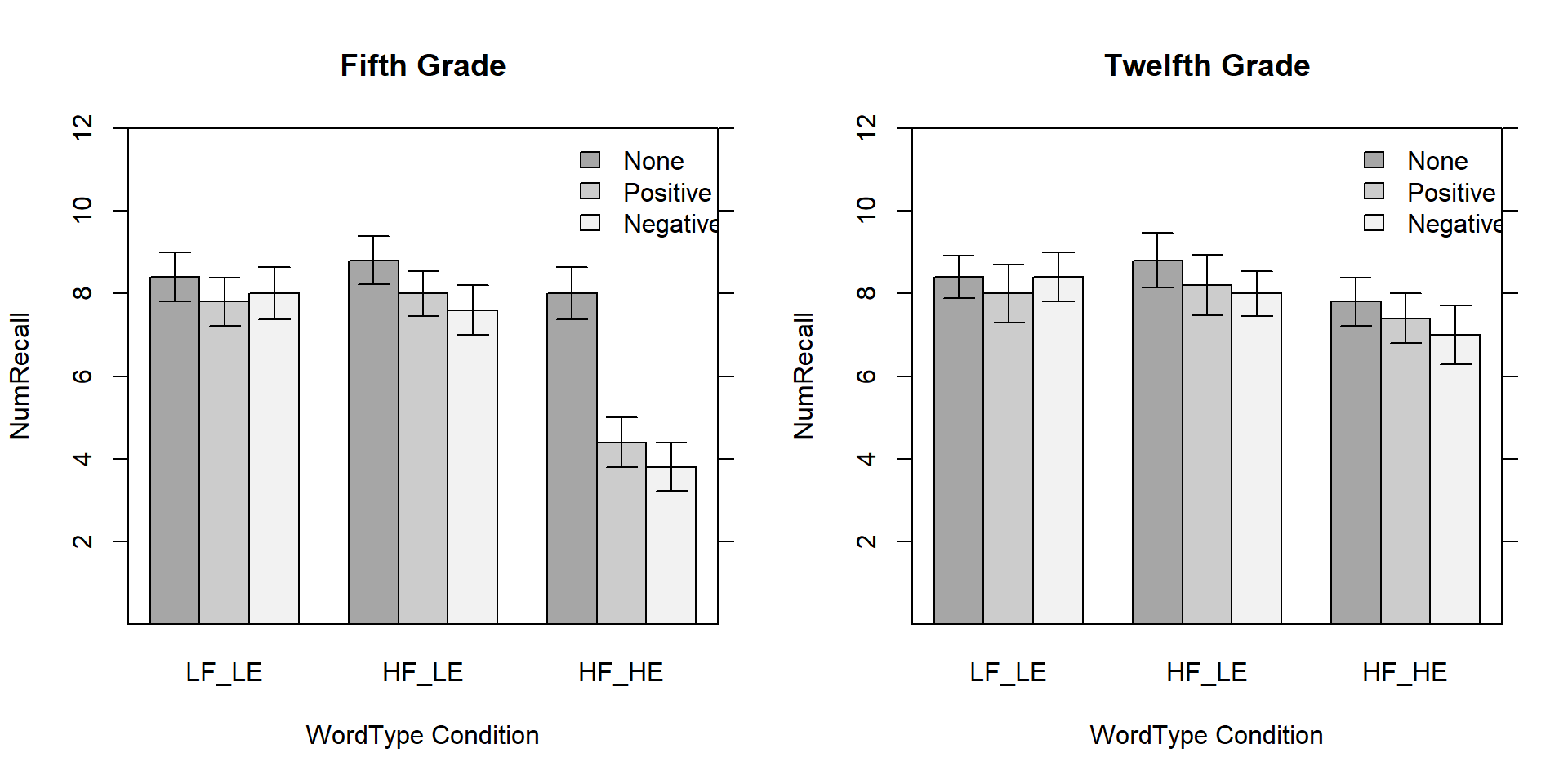``close.screen(all = TRUE) ``

### 2.4.3 Bar Graphs with ggplot2

With ggplot2 we have to produce an object that summarizes the data frame firts - extracting means and std errors. I did this with an Rmisc function.

``````# first, summarize the data set to produce a new data frame that ggplot can use
myData <- Rmisc::summarySE(data=bg.3way,measurevar="numrecall", groupvars=c("feedback", "wordtype", "grade"))
# look at the new data frame that contains the summary statistics
myData``````
feedback wordtype grade N numrecall sd se ci
None LF_LE fifth 5 8.4 1.341641 0.6000000 1.665867
None LF_LE twelfth 5 8.4 1.140175 0.5099020 1.415715
None HF_LE fifth 5 8.8 1.303840 0.5830952 1.618932
None HF_LE twelfth 5 8.8 1.483240 0.6633250 1.841685
None HF_HE fifth 5 8.0 1.414214 0.6324555 1.755978
None HF_HE twelfth 5 7.8 1.303840 0.5830952 1.618932
Positive LF_LE fifth 5 7.8 1.303840 0.5830952 1.618932
Positive LF_LE twelfth 5 8.0 1.581139 0.7071068 1.963243
Positive HF_LE fifth 5 8.0 1.224745 0.5477226 1.520722
Positive HF_LE twelfth 5 8.2 1.643168 0.7348469 2.040262
Positive HF_HE fifth 5 4.4 1.341641 0.6000000 1.665867
Positive HF_HE twelfth 5 7.4 1.341641 0.6000000 1.665867
Negative LF_LE fifth 5 8.0 1.414214 0.6324555 1.755978
Negative LF_LE twelfth 5 8.4 1.341641 0.6000000 1.665867
Negative HF_LE fifth 5 7.6 1.341641 0.6000000 1.665867
Negative HF_LE twelfth 5 8.0 1.224745 0.5477226 1.520722
Negative HF_HE fifth 5 3.8 1.303840 0.5830952 1.618932
Negative HF_HE twelfth 5 7.0 1.581139 0.7071068 1.963243

Now, use the myData object to draw the base plot (p1), and then add style control elements.

``````#library(ggplot2)
#library(ggthemes)
# Now create the Default bar plot
p1 <- ggplot(myData, aes(x=feedback, y=numrecall, fill=wordtype)) +
geom_bar(stat="identity", color="black",
position=position_dodge()) +
geom_errorbar(aes(ymin=numrecall-se, ymax=numrecall+se), width=.2,
position=position_dodge(.9)) +

p2 <- p1  +labs(title="Words Recalled +/- SEM", x="Feedback Group", y = "Mean Number Recalled")+
theme_bw() +
theme(panel.grid.major.x = element_blank(),
panel.grid.major.y = element_blank(),
panel.grid.minor.x = element_blank(),
panel.grid.minor.y = element_blank(),
panel.background = element_blank(),
axis.line.y = element_line(colour="black", size=.7),
axis.line.x = element_line(colour="black", size=.7),
plot.title = element_text(hjust=.5)
) +
scale_fill_manual(values=c('honeydew3','honeydew2', 'honeydew1')) ``````
``````## Warning: The `size` argument of `element_line()` is deprecated as of ggplot2 3.4.0.
``print(p2)``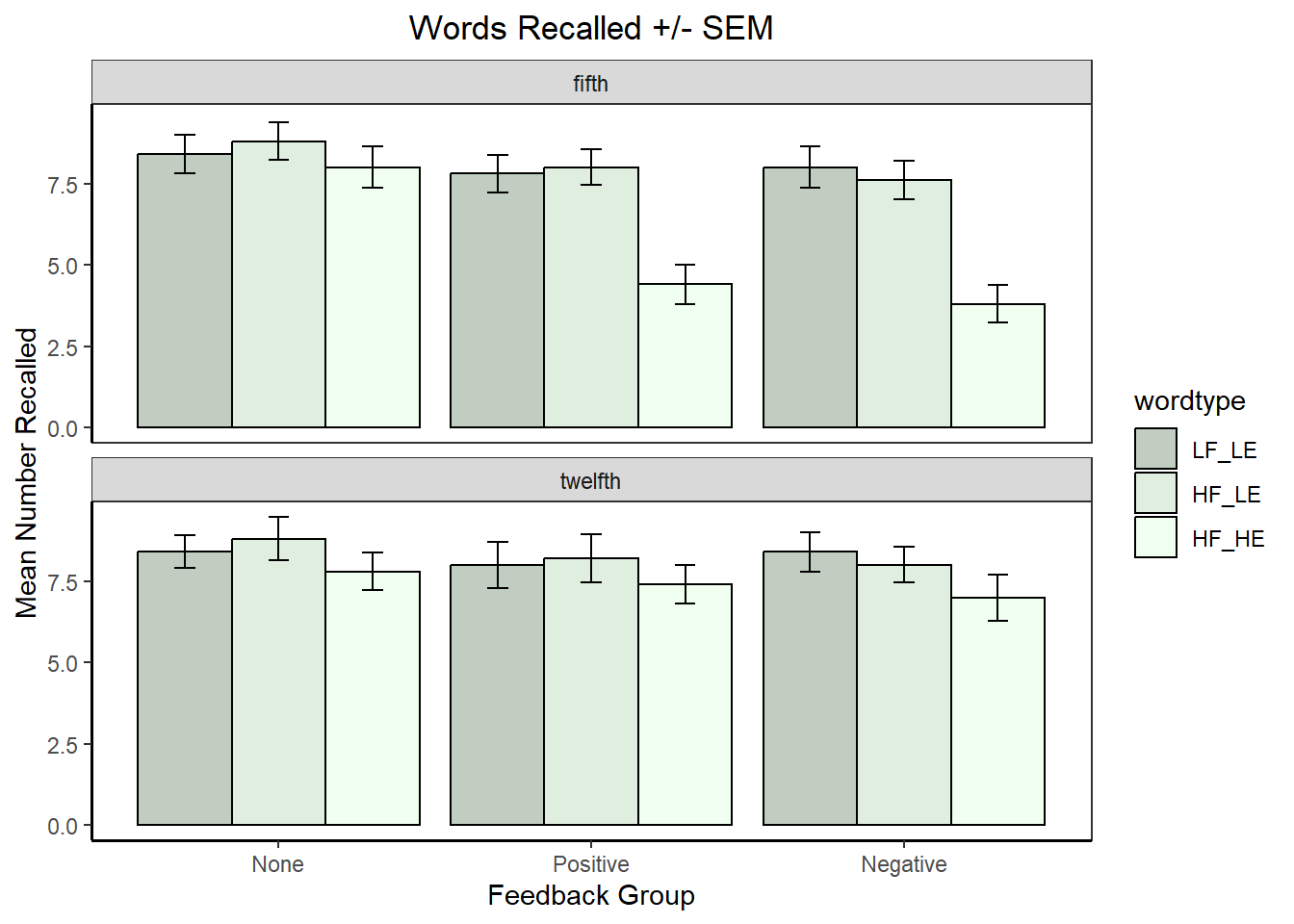# 3 Perform the basic/omnibus 3 way ANOVA

First, detach the attached data frame since `aov` permits specification of the data frame name.

``detach(bg.3way)``

## 3.1 Use the `aov` function for the base omnibus analysis

Fitting the core model with `aov` follows a similar logic to that used for 2-way designs. The model formula could have been written the following way:

``````# code not run
fit_base.aov <- aov(numrecall~feedback + wordtype + grade +
feedback:wordtype +
data=bg.3way)``````

The model syntax shown above, naming each effect in the model, is inefficient when we want a full factorial analysis. It is more efficient to use the asterisk operator which tells `aov` to create all higher order terms from the main effects listed.

``fit_base.aov <- aov(numrecall~feedback*wordtype*grade,data=bg.3way)``

### 3.1.1 a different way to obtain tables of means and std errors

This is an efficient function, but care must be taken in unbalanced designs to be certain of whether it produces weighted or unweighted marginal means. In our example this doesn’t matter since sample sizes are balanced.

``model.tables(fit_base.aov,"means",se=T)``
``````## Tables of means
## Grand mean
##
## 7.6
##
##  feedback
## feedback
##     None Positive Negative
##    8.367    7.300    7.133
##
##  wordtype
## wordtype
## LF_LE HF_LE HF_HE
## 8.167 8.233 6.400
##
##   fifth twelfth
##     7.2     8.0
##
##  feedback:wordtype
##           wordtype
## feedback   LF_LE HF_LE HF_HE
##   None     8.4   8.8   7.9
##   Positive 7.9   8.1   5.9
##   Negative 8.2   7.8   5.4
##
## feedback   fifth twelfth
##   None     8.400 8.333
##   Positive 6.733 7.867
##   Negative 6.467 7.800
##
## wordtype fifth twelfth
##    LF_LE 8.067 8.267
##    HF_LE 8.133 8.333
##    HF_HE 5.400 7.400
##
## , , grade = fifth
##
##           wordtype
## feedback   LF_LE HF_LE HF_HE
##   None     8.4   8.8   8.0
##   Positive 7.8   8.0   4.4
##   Negative 8.0   7.6   3.8
##
## , , grade = twelfth
##
##           wordtype
## feedback   LF_LE HF_LE HF_HE
##   None     8.4   8.8   7.8
##   Positive 8.0   8.2   7.4
##   Negative 8.4   8.0   7.0
##
##
## Standard errors for differences of means
##           0.3549   0.3549 0.2897            0.6146         0.5018
## replic.       30       30     45                10             15
##                 0.5018                  0.8692
## replic.             15                       5``````

### 3.1.2 Obtain ANOVA summary tables

The `summary` and `anova` functions produce the same summary table (Type I SS).

``summary(fit_base.aov)``
``````##                         Df Sum Sq Mean Sq F value   Pr(>F)
## feedback                 2  26.87   13.43   7.112  0.00152 **
## wordtype                 2  64.87   32.43  17.171 7.99e-07 ***
## grade                    1  14.40   14.40   7.624  0.00730 **
## feedback:wordtype        4  14.67    3.67   1.941  0.11283
## feedback:grade           2   8.60    4.30   2.276  0.10999
## wordtype:grade           2  16.20    8.10   4.288  0.01740 *
## feedback:wordtype:grade  4  10.00    2.50   1.324  0.26946
## Residuals               72 136.00    1.89
## ---
## Signif. codes:  0 '***' 0.001 '**' 0.01 '*' 0.05 '.' 0.1 ' ' 1``````
``anova(fit_base.aov)``
Df Sum Sq Mean Sq F value Pr(>F)
feedback 2 26.86667 13.433333 7.111765 0.0015186
wordtype 2 64.86667 32.433333 17.170588 0.0000008
grade 1 14.40000 14.400000 7.623529 0.0073041
feedback:wordtype 4 14.66667 3.666667 1.941177 0.1128292
feedback:grade 2 8.60000 4.300000 2.276471 0.1099872
wordtype:grade 2 16.20000 8.100000 4.288235 0.0173970
feedback:wordtype:grade 4 10.00000 2.500000 1.323529 0.2694608
Residuals 72 136.00000 1.888889 NA NA

### 3.1.3 Refit the model using `lm`

It is worth recalling that since `aov` is a wrapper for `lm`, we can we refit the model, using the `lm` function. This produces the same anova summary table as when we used `aov` above, so the residuals are the same. This approach will become useful when we examine analysis of contrasts, in unequal N designs.

``````# do the 3 way anova with the lm function
#summary(fit.1lm)
anova(fit.1lm)``````
Df Sum Sq Mean Sq F value Pr(>F)
feedback 2 26.86667 13.433333 7.111765 0.0015186
wordtype 2 64.86667 32.433333 17.170588 0.0000008
grade 1 14.40000 14.400000 7.623529 0.0073041
feedback:wordtype 4 14.66667 3.666667 1.941177 0.1128292
feedback:grade 2 8.60000 4.300000 2.276471 0.1099872
wordtype:grade 2 16.20000 8.100000 4.288235 0.0173970
feedback:wordtype:grade 4 10.00000 2.500000 1.323529 0.2694608
Residuals 72 136.00000 1.888889 NA NA

## 3.2 Use the afex package for the omnibus ANOVA

An alternative the provides some advantages is the suite of ANOVA tools from afex.

``````fit_base.afex <- aov_car(numrecall~feedback*wordtype*grade + Error(1|snum), data=bg.3way)
gt::gt(nice(fit_base.afex))``````
Effect df MSE F ges p.value
feedback 2, 72 1.89 7.11 ** .165 .002
wordtype 2, 72 1.89 17.17 *** .323 <.001
grade 1, 72 1.89 7.62 ** .096 .007
feedback:wordtype 4, 72 1.89 1.94 .097 .113
feedback:grade 2, 72 1.89 2.28 .059 .110
wordtype:grade 2, 72 1.89 4.29 * .106 .017
feedback:wordtype:grade 4, 72 1.89 1.32 .068 .269

# 4 Evaluate Assumptions

The normality and homoscedasticity/homogeneity assumptions are evaluated in the standard manner we covered for linear models and ANOVA objects.

## 4.1 First, produce the diagnostic plots for linear models

The standard two plots for evaluating homoscedasticity and normality of the residuals are available in the same manner as for other linear model objects that we have seen previously.

``plot(fit_base.aov,which=1)``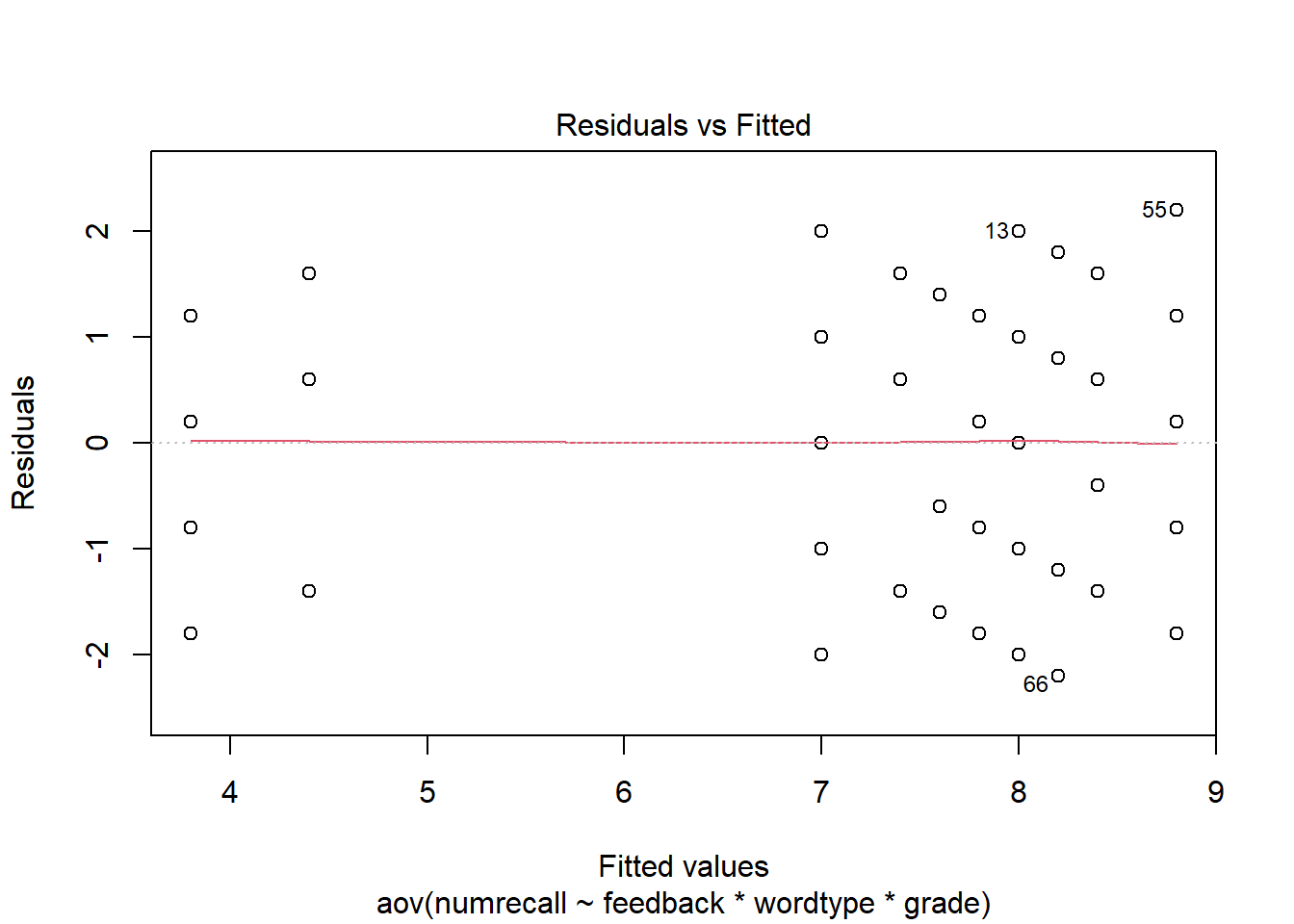``plot(fit_base.aov,which=2)``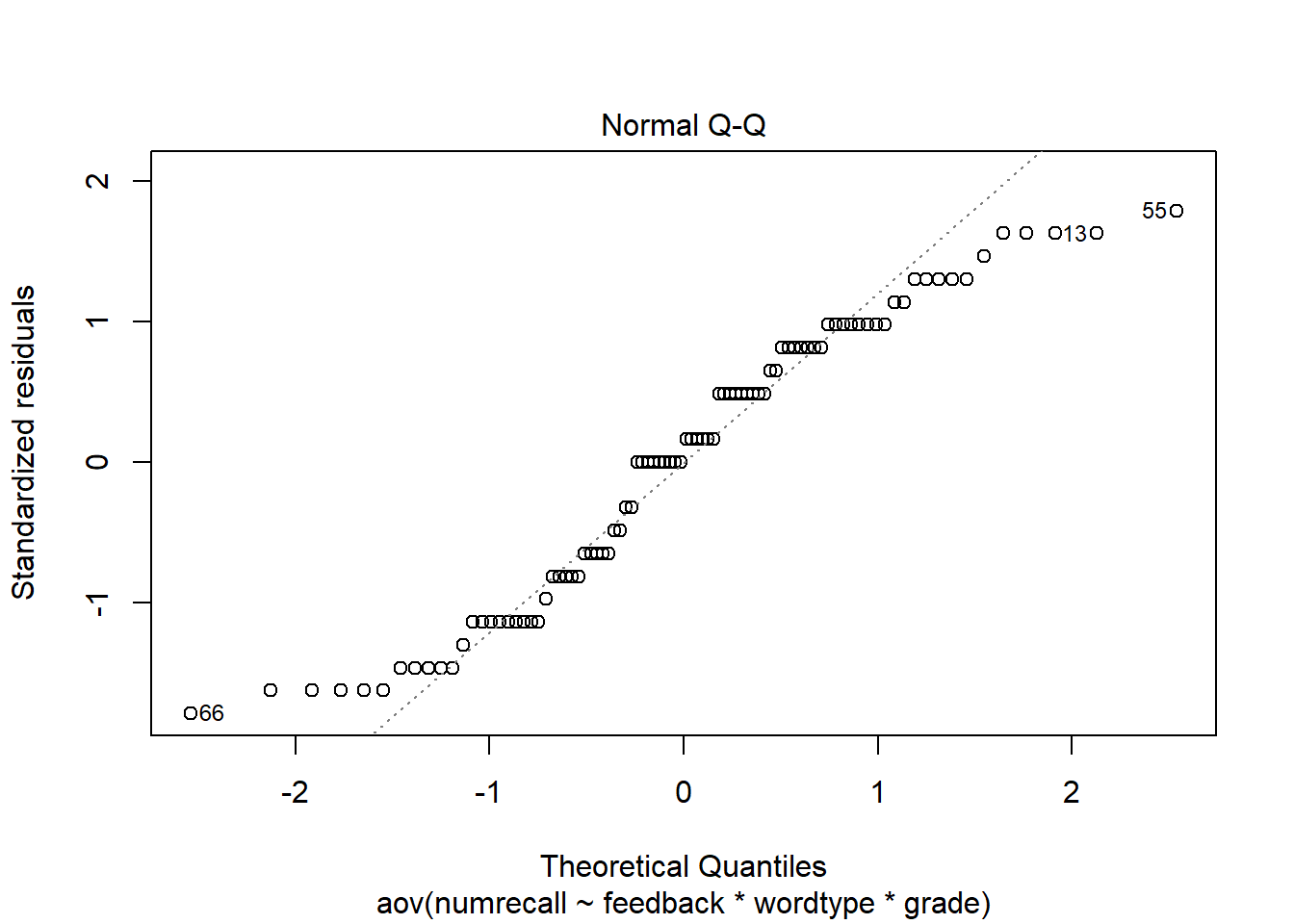The `qqPlot` function from the car package produces a nice normal QQ plot.

``car::qqPlot(fit_base.aov\$residuals)``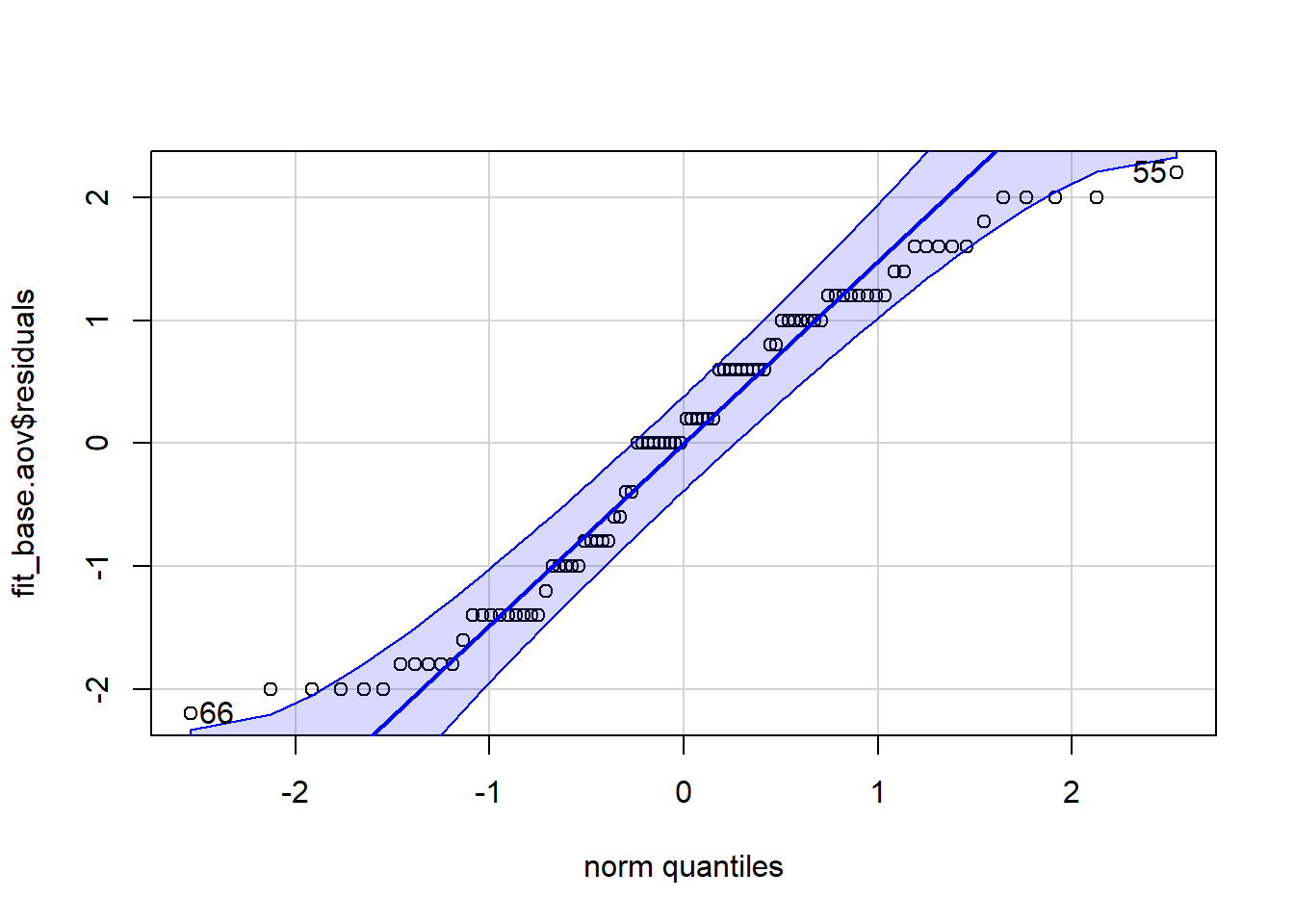``##  55 66``

There is clearly some non-normality in the distribution of these residuals so let’s examine a frequency histogram with a kernel denisity overlaid.

``````hist(fit_base.aov\$residuals, breaks=10, prob=TRUE)
lines(density(fit_base.aov\$residuals))``````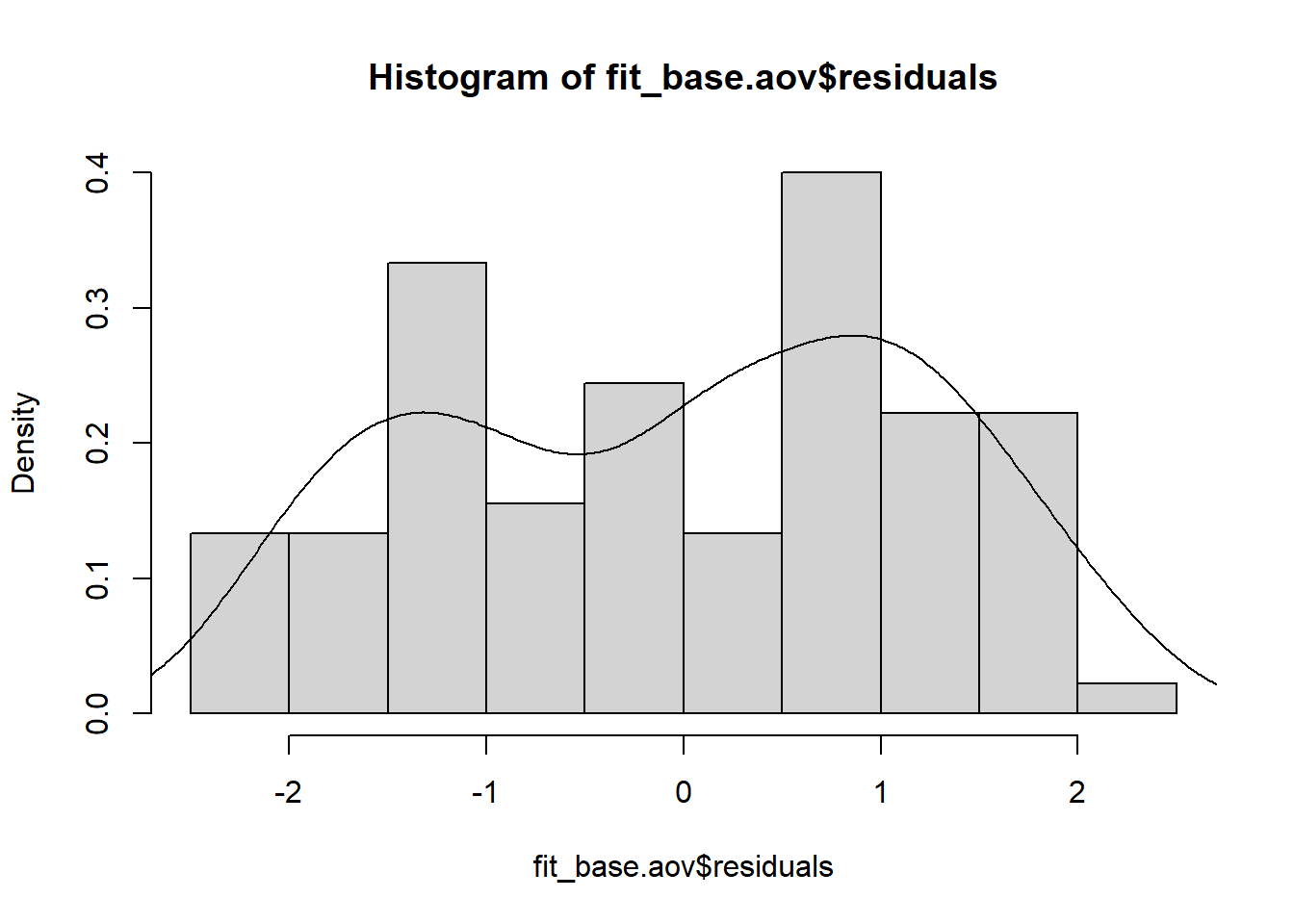## 4.2 Inferential tests of the Normality and Homogeneity of Variance assumptions

We can see some non-normality of the residuals from the graphical displays, so inferential tests might be in order. The Anderson-Darling test rejects the null hypothesis that residuals are normally distributed.

``nortest::ad.test(residuals(fit_base.aov))``
``````##
##  Anderson-Darling normality test
##
## data:  residuals(fit_base.aov)
## A = 1.5133, p-value = 0.0006257``````

A Shapiro-Wilk test reaches the same conclusion.

``shapiro.test(residuals(fit_base.aov))``
``````##
##  Shapiro-Wilk normality test
##
## data:  residuals(fit_base.aov)
## W = 0.94663, p-value = 0.001042``````

The Levene test for homogeneity of variance is obtained using the function from the car package. Very little variation in cell variances/stdevs was seen and this test was not significant.

``car::leveneTest(fit_base.aov)``
Df F value Pr(>F)
group 17 0.08967 0.9999992
72 NA NA

The Breusch Pagan test of homoscedasticity leads to a similar outcome, as does the non-constant variance test.

``lmtest::bptest(fit_base.aov)``
``````##
##  studentized Breusch-Pagan test
##
## data:  fit_base.aov
## BP = 4.1174, df = 17, p-value = 0.9994``````
``car::ncvTest(fit.1lm)``
``````## Non-constant Variance Score Test
## Variance formula: ~ fitted.values
## Chisquare = 0.008655078, Df = 1, p = 0.92588``````

# 5 Orthogonal Contrasts on the Omnibus Effects

We will obtain partitioning of omnibus effects into their single df contrasts and later use the emmeans and phia package both for these omnibus effects and to obtain simple effects.

## 5.1 Work with the `split` argument and the `summary.lm` function to obtain contrasts on omnibus effects.

The Feedback and Wordtype factors both have three levels and are now decomposed into a pair of orthogonal contrasts each. For Feedback, the first contrast compares the negative condition to the average of the other two and the second contrasts compares none and positive.

``````contrasts.feedback <- matrix(c(-1,-1,2,-1,1,0),ncol=2)
contrasts(bg.3way\$feedback) <- contrasts.feedback
contrasts(bg.3way\$feedback)``````
``````##          [,1] [,2]
## None       -1   -1
## Positive   -1    1
## Negative    2    0``````

For the Wordtype variable, the first contrast compares LF_LE to the other two, and the second compares HF-LE to HF_HE.

``````contrasts.wordtype <- matrix(c(2,-1,-1,0,-1,1),ncol=2)
contrasts(bg.3way\$wordtype) <- contrasts.wordtype
contrasts(bg.3way\$wordtype)``````
``````##       [,1] [,2]
## LF_LE    2    0
## HF_LE   -1   -1
## HF_HE   -1    1``````

I will redo the basic omnibus ANOVA here and the use the `split` function to obtain tests of contrasts.

``````fit.1aov <- aov(numrecall~feedback*wordtype*grade,data=bg.3way)
#summary(fit.1aov)
anova(fit.1aov)``````
Df Sum Sq Mean Sq F value Pr(>F)
feedback 2 26.86667 13.433333 7.111765 0.0015186
wordtype 2 64.86667 32.433333 17.170588 0.0000008
grade 1 14.40000 14.400000 7.623529 0.0073041
feedback:wordtype 4 14.66667 3.666667 1.941177 0.1128292
feedback:grade 2 8.60000 4.300000 2.276471 0.1099872
wordtype:grade 2 16.20000 8.100000 4.288235 0.0173970
feedback:wordtype:grade 4 10.00000 2.500000 1.323529 0.2694608
Residuals 72 136.00000 1.888889 NA NA

The initial application of this approach includes partitioning of both the Feedback and Wordtype factors. Grade is only two levels, so requires no further decomposition. Notice that `summary` now produces all eleven single df contrasts among the twelve cells, breaking down main effects and interactions involving Feedback and Wordtype.

``````summary(fit.1aov,  split=list(feedback=list(feedback.ac1=1, feedback.ac2=2),
wordtype=list(wordtype.ac1=1, wordtype.ac2=2)))``````
``````##                                                      Df Sum Sq Mean Sq F value
## feedback                                              2  26.87   13.43   7.112
##   feedback: feedback.ac1                              1   9.80    9.80   5.188
##   feedback: feedback.ac2                              1  17.07   17.07   9.035
## wordtype                                              2  64.87   32.43  17.171
##   wordtype: wordtype.ac1                              1  14.45   14.45   7.650
##   wordtype: wordtype.ac2                              1  50.42   50.42  26.691
## grade                                                 1  14.40   14.40   7.624
## feedback:wordtype                                     4  14.67    3.67   1.941
##   feedback:wordtype: feedback.ac1.wordtype.ac1        1   5.63    5.63   2.978
##   feedback:wordtype: feedback.ac2.wordtype.ac1        1   2.41    2.41   1.275
##   feedback:wordtype: feedback.ac1.wordtype.ac2        1   2.41    2.41   1.275
##   feedback:wordtype: feedback.ac2.wordtype.ac2        1   4.23    4.23   2.237
## feedback:grade                                        2   8.60    4.30   2.276
##   feedback:grade: feedback.ac1                        1   3.20    3.20   1.694
##   feedback:grade: feedback.ac2                        1   5.40    5.40   2.859
## wordtype:grade                                        2  16.20    8.10   4.288
##   wordtype:grade: wordtype.ac1                        1   4.05    4.05   2.144
##   wordtype:grade: wordtype.ac2                        1  12.15   12.15   6.432
## feedback:wordtype:grade                               4  10.00    2.50   1.324
##   feedback:wordtype:grade: feedback.ac1.wordtype.ac1  1   0.63    0.63   0.331
##   feedback:wordtype:grade: feedback.ac2.wordtype.ac1  1   1.88    1.88   0.993
##   feedback:wordtype:grade: feedback.ac1.wordtype.ac2  1   1.88    1.88   0.993
##   feedback:wordtype:grade: feedback.ac2.wordtype.ac2  1   5.62    5.62   2.978
## Residuals                                            72 136.00    1.89
##                                                        Pr(>F)
## feedback                                              0.00152 **
##   feedback: feedback.ac1                              0.02571 *
##   feedback: feedback.ac2                              0.00364 **
## wordtype                                             7.99e-07 ***
##   wordtype: wordtype.ac1                              0.00721 **
##   wordtype: wordtype.ac2                             2.05e-06 ***
## feedback:wordtype                                     0.11283
##   feedback:wordtype: feedback.ac1.wordtype.ac1        0.08870 .
##   feedback:wordtype: feedback.ac2.wordtype.ac1        0.26258
##   feedback:wordtype: feedback.ac1.wordtype.ac2        0.26258
##   feedback:wordtype: feedback.ac2.wordtype.ac2        0.13913
## Residuals
## ---
## Signif. codes:  0 '***' 0.001 '**' 0.01 '*' 0.05 '.' 0.1 ' ' 1``````

If we only pass one variable at a time to the `split` function, the resulting ANOVA table yields interaction comparisons instead of interaction contrasts.

``summary(fit.1aov,  split=list(feedback=list(feedback.ac1=1, feedback.ac2=2)))``
``````##                                         Df Sum Sq Mean Sq F value   Pr(>F)
## feedback                                 2  26.87   13.43   7.112  0.00152 **
##   feedback: feedback.ac1                 1   9.80    9.80   5.188  0.02571 *
##   feedback: feedback.ac2                 1  17.07   17.07   9.035  0.00364 **
## wordtype                                 2  64.87   32.43  17.171 7.99e-07 ***
## grade                                    1  14.40   14.40   7.624  0.00730 **
## feedback:wordtype                        4  14.67    3.67   1.941  0.11283
##   feedback:wordtype: feedback.ac1        2   8.03    4.02   2.126  0.12669
##   feedback:wordtype: feedback.ac2        2   6.63    3.32   1.756  0.18007
## feedback:grade                           2   8.60    4.30   2.276  0.10999
##   feedback:grade: feedback.ac1           1   3.20    3.20   1.694  0.19721
##   feedback:grade: feedback.ac2           1   5.40    5.40   2.859  0.09520 .
## wordtype:grade                           2  16.20    8.10   4.288  0.01740 *
## feedback:wordtype:grade                  4  10.00    2.50   1.324  0.26946
##   feedback:wordtype:grade: feedback.ac1  2   2.50    1.25   0.662  0.51905
##   feedback:wordtype:grade: feedback.ac2  2   7.50    3.75   1.985  0.14479
## Residuals                               72 136.00    1.89
## ---
## Signif. codes:  0 '***' 0.001 '**' 0.01 '*' 0.05 '.' 0.1 ' ' 1``````

Similarly, with only Wordtype partitioned…….

``summary(fit.1aov,  split=list(wordtype=list(wordtype.ac1=1, wordtype.ac2=2)))``
``````##                                         Df Sum Sq Mean Sq F value   Pr(>F)
## feedback                                 2  26.87   13.43   7.112  0.00152 **
## wordtype                                 2  64.87   32.43  17.171 7.99e-07 ***
##   wordtype: wordtype.ac1                 1  14.45   14.45   7.650  0.00721 **
##   wordtype: wordtype.ac2                 1  50.42   50.42  26.691 2.05e-06 ***
## grade                                    1  14.40   14.40   7.624  0.00730 **
## feedback:wordtype                        4  14.67    3.67   1.941  0.11283
##   feedback:wordtype: wordtype.ac1        2   8.03    4.02   2.126  0.12669
##   feedback:wordtype: wordtype.ac2        2   6.63    3.32   1.756  0.18007
## feedback:grade                           2   8.60    4.30   2.276  0.10999
## wordtype:grade                           2  16.20    8.10   4.288  0.01740 *
##   wordtype:grade: wordtype.ac1           1   4.05    4.05   2.144  0.14747
##   wordtype:grade: wordtype.ac2           1  12.15   12.15   6.432  0.01338 *
## feedback:wordtype:grade                  4  10.00    2.50   1.324  0.26946
##   feedback:wordtype:grade: wordtype.ac1  2   2.50    1.25   0.662  0.51905
##   feedback:wordtype:grade: wordtype.ac2  2   7.50    3.75   1.985  0.14479
## Residuals                               72 136.00    1.89
## ---
## Signif. codes:  0 '***' 0.001 '**' 0.01 '*' 0.05 '.' 0.1 ' ' 1``````

### 5.1.1 A WORD OF CAUTION

Please recall from work in the 2-way design and the oneway tutorial that use of this `split` function, while fairly simple, produces Type I SS decompositions when sample sizes are unequal. This may not be desirable, so other approaches are necessary. One is shown here, others are outlined below using the emmeans or phia packages.

When we apply the `summary.lm` function to an `aov` object, the resultant table is a table of the regression coefficients for the model. The t-tests here are tantamount to tests of Type III SS and may be more desirable when sample sizes are unequal. In our example, the squares of these t values equal the F tests from the summary tables above where `split` was use. But that was ONLY because sample sizes were equal in this example.

``summary.lm(fit.1aov)``
``````##
## Call:
## aov(formula = numrecall ~ feedback * wordtype * grade, data = bg.3way)
##
## Residuals:
##    Min     1Q Median     3Q    Max
##   -2.2   -1.0    0.1    1.0    2.2
##
## Coefficients:
##                                  Estimate Std. Error t value Pr(>|t|)
## (Intercept)                       7.20000    0.20488  35.143  < 2e-16 ***
## feedback1                        -0.36667    0.14487  -2.531  0.01356 *
## feedback2                        -0.83333    0.25092  -3.321  0.00141 **
## wordtype1                         0.43333    0.14487   2.991  0.00380 **
## wordtype2                        -1.36667    0.25092  -5.447 6.79e-07 ***
## gradetwelfth                      0.80000    0.28974   2.761  0.00730 **
## feedback1:wordtype1               0.16667    0.10244   1.627  0.10811
## feedback2:wordtype1               0.26667    0.17743   1.503  0.13723
## feedback1:wordtype2              -0.26667    0.17743  -1.503  0.13723
## feedback2:wordtype2              -0.70000    0.30732  -2.278  0.02571 *
## feedback1:gradetwelfth            0.26667    0.20488   1.302  0.19721
## feedback2:gradetwelfth            0.60000    0.35486   1.691  0.09520 .
## wordtype1:gradetwelfth           -0.30000    0.20488  -1.464  0.14747
## wordtype2:gradetwelfth            0.90000    0.35486   2.536  0.01338 *
## feedback1:wordtype1:gradetwelfth -0.08333    0.14487  -0.575  0.56693
## feedback2:wordtype1:gradetwelfth -0.25000    0.25092  -0.996  0.32243
## feedback1:wordtype2:gradetwelfth  0.25000    0.25092   0.996  0.32243
## feedback2:wordtype2:gradetwelfth  0.75000    0.43461   1.726  0.08870 .
## ---
## Signif. codes:  0 '***' 0.001 '**' 0.01 '*' 0.05 '.' 0.1 ' ' 1
##
## Residual standard error: 1.374 on 72 degrees of freedom
## Multiple R-squared:  0.5336, Adjusted R-squared:  0.4235
## F-statistic: 4.846 on 17 and 72 DF,  p-value: 9.884e-07``````

# 6 Use of the phia package for contrasts and simple effects

Two different motivations exist for using either phia or **emmeans* in order to evaluate contrasts and simple effects. The first is that they produce inferential tests based on Type III SS decompositions. The second is that they are the only way I know to obtain simple effects.

In the particular data set we are working with here, the only higher order effect that was significant was the Wordtype by Grade interaction. This means that we would typically only follow up that effect by looking at simple main effects of one of those factors at levels of the other, as well as their interaction contrasts. We would also follow up with contrasts on the Feedback factor since its main effect was significant and no interactions with Feedback were significant.

However, the approach taken here is to demonstrate how the whole suite of effects can be obtained. Initially this document uses the phia package for these evaluations and makes extensive use of the `testInteractions` function from that package. The methods by “holm”, and “Hochberg” are possible for p value adjustments with contrasts.

## 6.1 Simple two way interactions

Simple two way interactions can also be obtained (three sets). We don’t expect to be interested in any of these since the three way interaction was not significant, but we might have had an a-priori hypothesis about one or more of them, so illustration is included for completeness.

Each of the rows of the tables represent tests of the simple two-way at that level of the factor at which the effects are examined (e.g.,feedback by grade at levels of grade in the first illustration).

The general strategy in the `testInteractions` function is to use an argument called “fixed” to specify the variable at which effects of other factors are to be examined in simple effects. The “across” argument specifies a variable for which the effect is being requested.

Note that the combined effects of two variables are requested by putting both of them in the “across” argument - the variable “AT” which these effects are examined is designated by the “fixed” argument.

Reading the table requires some understanding of the idiosyncracies of `testInteractions` formatting. The columns labeled (for example) feedback1:wordtype1 are not interactions as the colon implies. The numeric appendage (e.g., feedback1) means a contrast. The numeric values (e.g., 6, 3.2, etc) are “estimates” of the underlying contrast. But since we are not examining contrasts here we can ignore these columns. The F tests are tests of the two different simple two way interactions at the two levels of grade. At least the question of level of grade is clear in the table - rows.

``````# next, feedback*wordtype at levels of grade
feedback1:wordtype1 feedback2:wordtype1 feedback1:wordtype2 feedback2:wordtype2 Df Sum of Sq F Pr(>F)
fifth 6 3.2 -3.2 -2.8 4 23.333333 3.0882353 0.0209781
twelfth 3 0.2 -0.2 0.2 4 1.333333 0.1764706 0.9498252
Residuals NA NA NA NA 72 136.000000 NA NA

A second possible set of simple two way interactions is found here, wordtype by grade at levels of feedback.

``````# first wordtype*grade at levels of feedback
wordtype1 wordtype2 Df Sum of Sq F Pr(>F)
None -0.2 0.2 2 0.0666667 0.0176471 0.9825120
Positive 2.8 -2.8 2 13.0666667 3.4588235 0.0367876
Negative 2.8 -2.8 2 13.0666667 3.4588235 0.0367876
Residuals NA NA 72 136.0000000 NA NA

And now the third set:

``````# next, feedback*grade at levels of wordtype
feedback1 feedback2 Df Sum of Sq F Pr(>F)
LF_LE -0.6 -0.2 2 0.2 0.0529412 0.9484727
HF_LE -0.6 -0.2 2 0.2 0.0529412 0.9484727
HF_HE -3.6 -3.2 2 18.2 4.8176471 0.0108733
Residuals NA NA 72 136.0 NA NA

The analyst would likely never do all three sets. One one should suffice and it would probably be the one where the two primary IVs were interacted. In our example, the first one fits the way the ggplot bar graph is drawn so that is probably what the choice would be.

## 6.2 Simple-simple main effects

These are effects of one factor at combined levels of two other factors.

First examine the so-called simple, simple main effects (three different sets). These effects would be assessed after finding significant simple two way interactions. Notice that the `testInteractions` function accepts an argument for p value adjustment (e.g., bonferroni/holm/fdr), but that I’ve set it to “none” in these illustrations.

The general strategy in the `testInteractions` function is to use an argument called “fixed” to specify the variable at which effects of other factors are to be examined in simple effects. The “across” argument specifies a variable for which the effect is being requested.

The first set here examines effects of wordtype at combinations of the other two factor levels and is one of the sets of simple simple main effects most visible from the ggplot bar graph above.

Here, and for later tables, “values” of effects are differentiated by the contrasts associated with the factors although those are aggregated to obtain the simple effects - seen since many of them have multiple df. We are ignoring those “effect” values here since we have not addressed the contrasts in place at the time the `aov` fit was done (default is dummy coding). The exact choice of contrast set doesn’t influence the 2 df simple simple main effects here.

Notice that each of these have 2 df (three means compared, so 2 df). Later we will break the effects down into contrasts. Compare the significance test results to the ggplot bar graph. The only significant simple simple main effects in this table are the wordtype comparisons in the upper panel (fifth grade) for the negative and positive feedback conditions. This pattern of significance fits what the eye sees in the graph.

``````# 1,  effects of wordtype @ combinations of feedback and grade
wordtype1 wordtype2 Df Sum of Sq F Pr(>F)
None : fifth 0.0 -0.8 2 1.600000 0.4235294 0.6563524
Positive : fifth 3.2 -3.6 2 40.933333 10.8352941 0.0000770
Negative : fifth 4.6 -3.8 2 53.733333 14.2235294 0.0000062
None : twelfth 0.2 -1.0 2 2.533333 0.6705882 0.5145724
Positive : twelfth 0.4 -0.8 2 1.733333 0.4588235 0.6338618
Negative : twelfth 1.8 -1.0 2 5.200000 1.3764706 0.2590309
Residuals NA NA 72 136.000000 NA NA

We could also look at other sets of simple simple main effects but would probably only look at one (such as the immediately preceding one), followed up by contrasts (later in this doc).

``````# 2,  effects of grade @ combinations of feedback and wordtype
Value Df Sum of Sq F Pr(>F)
None : LF_LE 0.0 1 0.0 0.0000000 1.0000000
Positive : LF_LE -0.2 1 0.1 0.0529412 0.8186747
Negative : LF_LE -0.4 1 0.4 0.2117647 0.6467745
None : HF_LE 0.0 1 0.0 0.0000000 1.0000000
Positive : HF_LE -0.2 1 0.1 0.0529412 0.8186747
Negative : HF_LE -0.4 1 0.4 0.2117647 0.6467745
None : HF_HE 0.2 1 0.1 0.0529412 0.8186747
Positive : HF_HE -3.0 1 22.5 11.9117647 0.0009371
Negative : HF_HE -3.2 1 25.6 13.5529412 0.0004450
Residuals NA 72 136.0 NA NA

And the third possible set of simple simple main effects:

``````# 3,  effects of feedback @ combinations of grade and wordtype
feedback1 feedback2 Df Sum of Sq F Pr(>F)
fifth : LF_LE -0.2 -0.6 2 0.9333333 0.2470588 0.7817542
twelfth : LF_LE 0.4 -0.4 2 0.5333333 0.1411765 0.8685758
fifth : HF_LE -1.6 -0.8 2 3.7333333 0.9882353 0.3772246
twelfth : HF_LE -1.0 -0.6 2 1.7333333 0.4588235 0.6338618
fifth : HF_HE -4.8 -3.6 2 51.6000000 13.6588235 0.0000094
twelfth : HF_HE -1.2 -0.4 2 1.6000000 0.4235294 0.6563524
Residuals NA NA 72 136.0000000 NA NA

## 6.3 Simple main effects

In studies where the 3way interaction is not significant, the analyst would progress to examining the omnibus 2way interactions. If any are significant then they would partly be characterized by examining simple main effects (there are six possible sets here, two each for each of the three possible 2way interactions).

It is not possible to interpret such simple main effects without reference to the tables of collapsed/marginal means such as those produce above following the initial `aov` fit OR be examining the redrawn graph that depicts 2way layouts, collapsed on one of the three factors.

Such a redrawn graph is presented here, to depict the two way layout of wordtype and feedback (a 3x3 structure), since the first of the six sets of SME shown here examines the effect of wordtype at levels of feedback. If this were actual data analysis of a true data set (rather than a textbook/artifical one), then we would only look to follow up the wordtype by grade two way interaction since it was the only one of the three that reached traditional significance levels. But this first graphical illustration permits seeing the more complex 3x3 arrangement, even though the wordtype by feedback interaction was NS. The reader should recognize that the effects of wordtype (seen in the plot below) appear to depend on level of feedback.

From this depiction, we might expect to find an effect of wordtype only in the negative and positive feedback conditions. In reality the 2way interaction tests whether the wordtype effect is different in the three levels of feedback. It was the general impression that the HF_HE group is lower only in the positive and negative feedback levels. Nontheless while this impression is clear, the differnece was not significant with the wordtype by feedback interaction was tested.

``````# first, summarize the data set to produce a new data frame that ggplot can use
myData2.wg <- Rmisc::summarySE(data=bg.3way,measurevar="numrecall", groupvars=c("feedback", "wordtype"))
# look at the new data frame that contains the summary statistics
myData2.wg``````
feedback wordtype N numrecall sd se ci
None LF_LE 10 8.4 1.173788 0.3711843 0.8396772
None HF_LE 10 8.8 1.316561 0.4163332 0.9418111
None HF_HE 10 7.9 1.286684 0.4068852 0.9204382
Positive LF_LE 10 7.9 1.370320 0.4333333 0.9802681
Positive HF_LE 10 8.1 1.370320 0.4333333 0.9802681
Positive HF_HE 10 5.9 2.024846 0.6403124 1.4484873
Negative LF_LE 10 8.2 1.316561 0.4163332 0.9418111
Negative HF_LE 10 7.8 1.229273 0.3887301 0.8793686
Negative HF_HE 10 5.4 2.170509 0.6863753 1.5526889
``````#library(ggplot2)
#library(ggthemes)
# Now create the Default bar plot
p3 <- ggplot(myData2.wg, aes(x=feedback, y=numrecall, fill=wordtype)) +
geom_bar(stat="identity", color="black",
position=position_dodge()) +
geom_errorbar(aes(ymin=numrecall-se, ymax=numrecall+se), width=.2,
position=position_dodge(.9))

p4 <- p3  +labs(title="Words Recalled +/- SEM", x="Feedback", y = "Mean Number Recalled")+
theme_bw() +
theme(panel.grid.major.x = element_blank(),
panel.grid.major.y = element_blank(),
panel.grid.minor.x = element_blank(),
panel.grid.minor.y = element_blank(),
panel.background = element_blank(),
axis.line.y = element_line(colour="black", size=.7),
axis.line.x = element_line(colour="black", size=.7),
plot.title = element_text(hjust=.5)
) +
scale_fill_manual(values=c('honeydew3','honeydew2', 'honeydew1'))
p4``````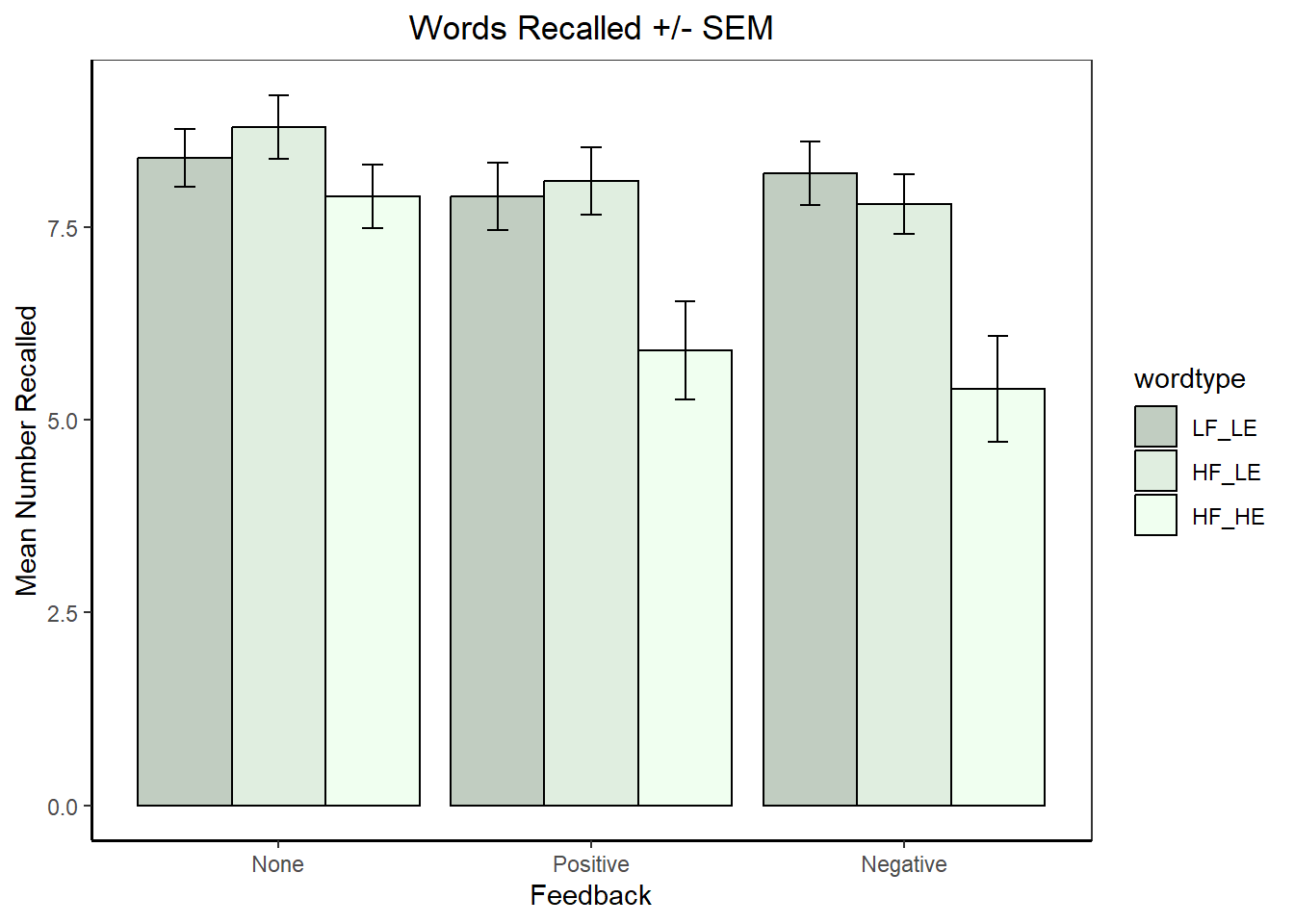The following sequence of tests of six sets of simple main effects is presented only to demonstrate how to generate each of them. We would probably only examine number 3 or 4.

In the first analysis we find that in the both the negative feedback condition the effect of feedback significant, as the graph seems to suggest. But note that most of the difference is due to the position of the HF_HE group. We would pursue that pattern with examination of simple main effect contrasts.

``````# 1-2  effects of wordtype at levels of feedback, and then feedback at levels of wordtype
# note these are collapsed on grade
wordtype1 wordtype2 Df Sum of Sq F Pr(>F)
None 0.1 -0.9 2 4.066667 1.076471 0.3462168
Positive 1.8 -2.2 2 29.600000 7.835294 0.0008341
Negative 3.2 -2.4 2 45.866667 12.141177 0.0000286
Residuals NA NA 72 136.000000 NA NA
``testInteractions(fit.1aov,fixed="wordtype", across="feedback",adjust="none")``
feedback1 feedback2 Df Sum of Sq F Pr(>F)
LF_LE 0.1 -0.5 2 1.266667 0.3352941 0.7162383
HF_LE -1.3 -0.7 2 5.266667 1.3941176 0.2546664
HF_HE -3.0 -2.0 2 35.000000 9.2647059 0.0002627
Residuals NA NA 72 136.000000 NA NA

Next we will begin to examine the origins of omnibus wordtype by grade interaction that was significant. In order to interpret the sets of simples possible in this 3x2 grid of means, we should visualize the outcome.

``````# first, summarize the data set to produce a new data frame that ggplot can use
# look at the new data frame that contains the summary statistics
myData3.wg``````
wordtype grade N numrecall sd se ci
LF_LE fifth 15 8.066667 1.279881 0.3304638 0.7087744
LF_LE twelfth 15 8.266667 1.279881 0.3304638 0.7087744
HF_LE fifth 15 8.133333 1.302013 0.3361783 0.7210308
HF_LE twelfth 15 8.333333 1.397276 0.3607752 0.7737858
HF_HE fifth 15 5.400000 2.292846 0.5920103 1.2697358
HF_HE twelfth 15 7.400000 1.352247 0.3491486 0.7488493
``````#library(ggplot2)
#library(ggthemes)
# Now create the Default bar plot
p5 <- ggplot(myData3.wg, aes(x=wordtype, y=numrecall, fill=grade)) +
geom_bar(stat="identity", color="black",
position=position_dodge()) +
geom_errorbar(aes(ymin=numrecall-se, ymax=numrecall+se), width=.2,
position=position_dodge(.9))

p6 <- p5  +labs(title="Words Recalled +/- SEM", x="WordType", y = "Mean Number Recalled")+
theme_bw() +
theme(panel.grid.major.x = element_blank(),
panel.grid.major.y = element_blank(),
panel.grid.minor.x = element_blank(),
panel.grid.minor.y = element_blank(),
panel.background = element_blank(),
axis.line.y = element_line(colour="black", size=.7),
axis.line.x = element_line(colour="black", size=.7),
plot.title = element_text(hjust=.5)
) +
scale_fill_manual(values=c('honeydew3','honeydew2', 'honeydew1'))
p6``````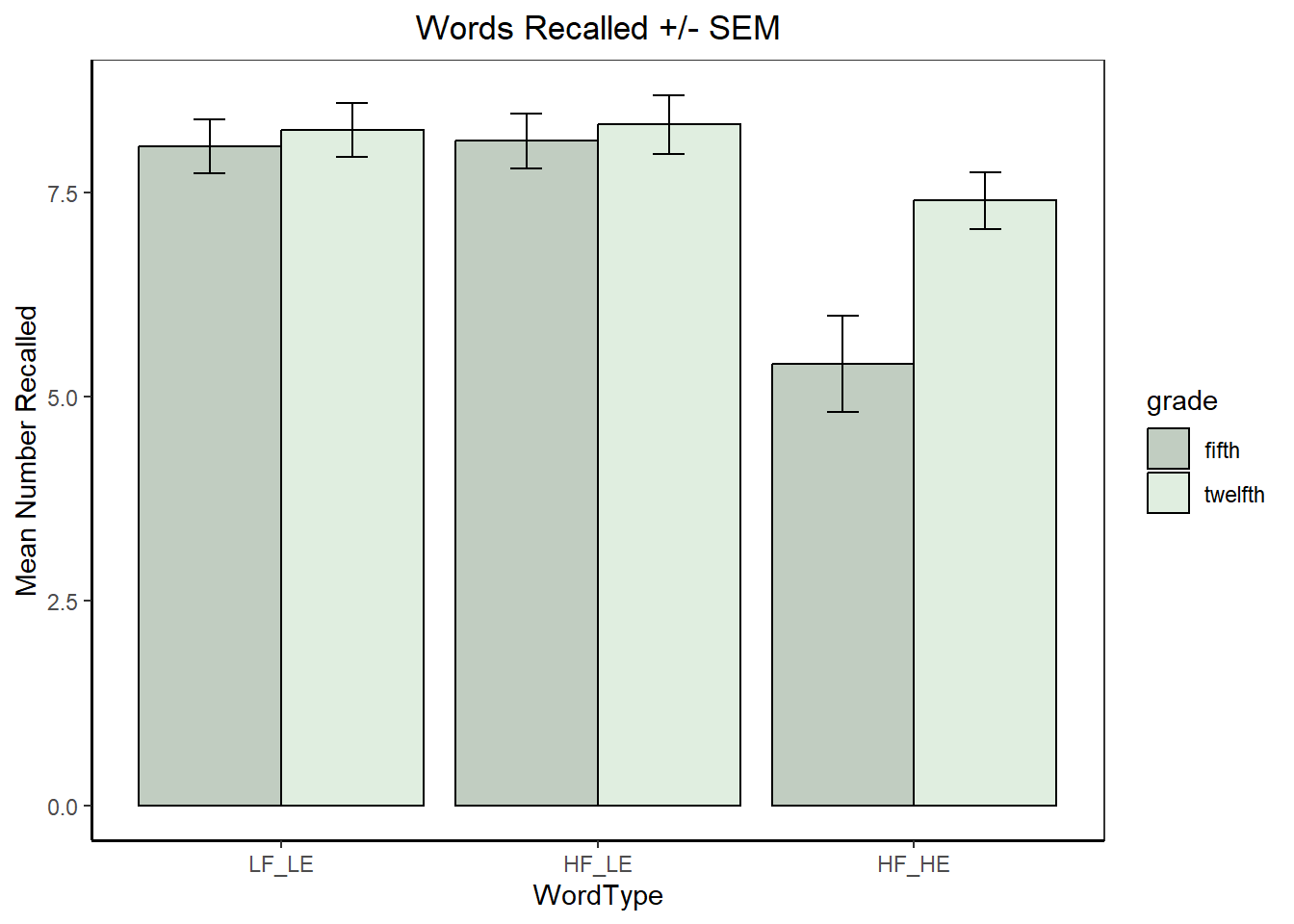In the first of the `testInteractions` analyses in this next code chunk (#3), the set of simple main effects of wordtype at levels of grade is produced. From the graph we may expect wordtype to be significant in the 12th graders, but perhaps not in the 5th graders. This is exactly what happened, as seen in the first table. In the second `testInteractions` output here (#4) the effect of grade is significant only in the HF_HE condition, as the visual inspection would have suggested.

``````# 3-4  effects of wordtype at levels of grade, and then grade at levels of feedback
# note these are collapsed on feedback, respectively
wordtype1 wordtype2 Df Sum of Sq F Pr(>F)
fifth 2.6 -2.7333333 2 72.933333 19.305882 0.0000002
twelfth 0.8 -0.9333333 2 8.133333 2.152941 0.1235609
Residuals NA NA 72 136.000000 NA NA
``testInteractions(fit.1aov,fixed="wordtype", across="grade",adjust="none")``
Value Df Sum of Sq F Pr(>F)
LF_LE -0.2 1 0.3 0.1588235 0.6914214
HF_LE -0.2 1 0.3 0.1588235 0.6914214
HF_HE -2.0 1 30.0 15.8823529 0.0001597
Residuals NA 72 136.0 NA NA

The third omnibus 2way can also be followed up with either of its set of simple main effects.

``````# 5-6  effects of feedback at levels of grade, and then grade at levels of feedback
# note these are collapsed on  wordtype
feedback1 feedback2 Df Sum of Sq F Pr(>F)
fifth -2.2 -1.6666667 2 32.933333 8.7176471 0.0004071
twelfth -0.6 -0.4666667 2 2.533333 0.6705882 0.5145724
Residuals NA NA 72 136.000000 NA NA
``testInteractions(fit.1aov,fixed="feedback", across="grade",adjust="none")``
Value Df Sum of Sq F Pr(>F)
None 0.0666667 1 0.0333333 0.0176471 0.8946887
Positive -1.1333333 1 9.6333333 5.1000000 0.0269569
Negative -1.3333333 1 13.3333333 7.0588235 0.0097084
Residuals NA 72 136.0000000 NA NA

## 6.4 Contrast Analyses in factorial designs using phia

Initially, we recreate the custom contrasts for the Wordtype and Feedback factors since they each have more than two levels. Note that these are the same contrasts employed above in the omnibus analyses, but phia requires them to be created in a slightly different manner for usage in the `testInteraction` function. The exact orthogonal sets employed here were arbitrarily chosen

``````# first for the feedback factor:
fbc1 <- list(feedback=c(-.5, -.5, 1)) # same as defined above, except using fractions
fbc2 <- list(feedback=c(-1, 1, 0)) # same as defined above, except using fractions
fbc1``````
``````## \$feedback
##  -0.5 -0.5  1.0``````
``fbc2``
``````## \$feedback
##  -1  1  0``````
``````# now for the wordtype factor
wtypec1 <- list(wordtype=c(1, -.5, -.5)) # same as defined above, except using fractions
wtypec2 <- list(wordtype=c(0, -1, 1)) # same as defined above, except using fractions
wtypec1``````
``````## \$wordtype
##   1.0 -0.5 -0.5``````
``wtypec2``
``````## \$wordtype
##   0 -1  1``````

### 6.4.1 Simple Effect Contrasts and Simple Interaction Contrasts

We already obtained 2 and 3-way interaction contrasts above with the summary/split approach and by using `summary.lm` to obtain Type III SS equivalents. Here, we obtain simple interaction contrasts and simple effect contrasts.

In this code, notice that `testInteractions` takes a “custom” argument and that is where we can specify the contrast to be used.

#### 6.4.1.1 Simple 2 way interaction contrasts

First we examine the simple two-way two way interactions (even though the 3way is NS) to serve as a template. NEEDS REWORDING Note that the labeling in the table is odd. The two`testInteraction` functions produce the two tables. The first examines the effect involving the first contrast on feedback (called fbc1 in the custom argument), and the second examines the second contrast on feedback (called fbc1). Examining the first of the two tables, each row represents evaluation of a simple two way interaction contrast of the Feedback (first contrast) and Grade at each of the three levels of Wordtype.

The use of the colon symbol does NOT imply an interaction between those two effects. In addition, the labeling of the Feedback effect as “feedback1” doesn’t refer to the exact contrast that we called fbc1. It is just a generic label for the fact that a contrast on feedback was specified. So in the first table, the first F value of .0794 represents a test of fbc1 by grade AT LF_LE. The second, is the same effect, but AT HF_LE and so forth. In the second table, the first row evaluates the fbc2 by grade interaction AT LF_LE, with the F value of .0265 EVEN THOUGH the table still uses the “feedback1” label. The confusion can arise because the tables do not employ the fbc1 and fbc2 labels that we created. In each table, there are three tests because there are three levels of Wordtype and the effect is either fbc1 by grade (table 1) or fbc2 by grade (table 2). This pattern plays out in subsequent implementations as well.

``````# first, an example of simple 2-way interaction contrasts of feedback with grade at levels of wordtype
Value Df Sum of Sq F Pr(>F)
LF_LE : feedback1 -0.3 1 0.15 0.0794118 0.7789045
HF_LE : feedback1 -0.3 1 0.15 0.0794118 0.7789045
HF_HE : feedback1 -1.8 1 5.40 2.8588235 0.0951989
Residuals NA 72 136.00 NA NA
``testInteractions(fit.1aov, fixed="wordtype", custom=fbc2, adjustment="none", across="grade")``
Value Df Sum of Sq F Pr(>F)
LF_LE : feedback1 -0.2 1 0.05 0.0264706 0.8712121
HF_LE : feedback1 -0.2 1 0.05 0.0264706 0.8712121
HF_HE : feedback1 -3.2 1 12.80 6.7764706 0.0112095
Residuals NA 72 136.00 NA NA

When both factors in a simple 2way interaction have contrasts then the code specification is slightly more complex. With the interaction of wordtype and feedback at levels of grade, grade is specified as “fixed”. The “across” argument is not used. Rather, both contrasts are specified simultaneously in the “custom” argument and `testInteractions understands that the interaction of those two contrasts is to be examined.

Note that the output uses the colon symbol again, but it doesn’t mean interaction. The interaction here is between fbc1 and wtypec1 (thus an interaction contrast) at fifth grade.

``````# simple 2-2way interaction contrast of wordtype and grade at levels of feedback
# one requested at a time here, both of the first contrasts (fbc1 and wtypec1) are requested.
Value Df Sum of Sq F Pr(>F)
fifth : feedback1 : wordtype1 1.50 1 5.00 2.6470588 0.1081106
twelfth : feedback1 : wordtype1 0.75 1 1.25 0.6617647 0.4186197
Residuals NA 72 136.00 NA NA

Code for the other six contrasts available in this set is shown here, but results suppressed to save space. If one were to run them the same “feedback1” and “wordtype1” labels would appear, but meaning either fbc1/fbc2 or wtypec1/wtypec2, respectively.

``````testInteractions(fit.1aov,fixed="grade", custom=c(fbc1,wtypec2), adjustment="none")

Sometimes we prefer to examine simple interaction comparisons rather than simple interaction contrasts. That kind of effect is exemplified with this type of code where a contrast is specified for one factor (wordtype here) and the other factor is not broken into contrasts (feedback here, specified in the “across” argument). These will be a 2 df interaction contrasts: either wordtype contrast 1 by feedback at levels of grade or wordtype contrast2 by feedback at levels of grade.

Once again the fifth: and twelfth: notation just specify the levels of grade AT which the simple interaction comparisons are located.

``````# now simple 2-way interaction comparisons of wordtype contrasts and feedback at levels of grade
# note that these effects have 2 df
feedback1 feedback2 Df Sum of Sq F Pr(>F)
fifth : wordtype1 3.0 1.6 2 9.266667 2.4529412 0.0932021
twelfth : wordtype1 1.5 0.1 2 1.266667 0.3352941 0.7162383
Residuals NA NA 72 136.000000 NA NA
``testInteractions(fit.1aov, fixed="grade", custom=wtypec2, adjustment="none", across="feedback")``
feedback1 feedback2 Df Sum of Sq F Pr(>F)
fifth : wordtype1 -3.2 -2.8 2 14.0666667 3.7235294 0.028918
twelfth : wordtype1 -0.2 0.2 2 0.0666667 0.0176471 0.982512
Residuals NA NA 72 136.0000000 NA NA

#### 6.4.1.2 Simple and simple-simple main effect contrasts

Contrasts breaking down the simple-simple or simple main effects are also available. Once again, care is required in reading the table, which does not label the different contrasts. The first table is for the fbc1 contrast and the second table for the fbc2 contrast, each at the six combined levels of Grade and Wordtype in this first illustration.

Once again, the “feedback1” label in the tables is misleading and not necessary. We have to discern which contrast on feedback is being tested by knowing which code line produced which table.

``````# Now, how about simple SME contrasts for the feedback factor at
# combined levels of grade and wordtype
Value Df Sum of Sq F Pr(>F)
LF_LE : fifth : feedback1 -0.1 1 0.0333333 0.0176471 0.8946887
HF_LE : fifth : feedback1 -0.8 1 2.1333333 1.1294118 0.2914524
HF_HE : fifth : feedback1 -2.4 1 19.2000000 10.1647059 0.0021193
LF_LE : twelfth : feedback1 0.2 1 0.1333333 0.0705882 0.7912415
HF_LE : twelfth : feedback1 -0.5 1 0.8333333 0.4411765 0.5086768
HF_HE : twelfth : feedback1 -0.6 1 1.2000000 0.6352941 0.4280406
Residuals NA 72 136.0000000 NA NA
``testInteractions(fit.1aov, fixed=c("wordtype", "grade"), custom=fbc2, adjustment="none")``
Value Df Sum of Sq F Pr(>F)
LF_LE : fifth : feedback1 -0.6 1 0.9 0.4764706 0.4922445
HF_LE : fifth : feedback1 -0.8 1 1.6 0.8470588 0.3604591
HF_HE : fifth : feedback1 -3.6 1 32.4 17.1529412 0.0000926
LF_LE : twelfth : feedback1 -0.4 1 0.4 0.2117647 0.6467745
HF_LE : twelfth : feedback1 -0.6 1 0.9 0.4764706 0.4922445
HF_HE : twelfth : feedback1 -0.4 1 0.4 0.2117647 0.6467745
Residuals NA 72 136.0 NA NA

Next, we evaluate Simple Main effect contrasts of Feedback at levels of Wordtype, collapsed on Grade. These next three code chunks also produce the two contrast tests with and without a “holm” adjustment.

The first code chunk is thus the test of feedback contrast 1 at levels of wordtype, with no p value adjustment. Once again, the “feedback1” label can be ignored. As an interpretation example, consider the third F test with a value of 7.94. This effect is feedback contrast 1 in the HF_HE group (collapsed on grade)

``````# or SME contrasts at levels of wordtype (collapsed on grade)
Value Df Sum of Sq F Pr(>F)
LF_LE : feedback1 0.05 1 0.0166667 0.0088235 0.9254228
HF_LE : feedback1 -0.65 1 2.8166667 1.4911765 0.2260177
HF_HE : feedback1 -1.50 1 15.0000000 7.9411765 0.0062342
Residuals NA 72 136.0000000 NA NA

The next chunk does the same analysis with a “holm” adjustment.

``````# note that adjustment for Type I error inflation can be done.  The "adjustment"
# argument uses the methods available in p.adjust
Value Df Sum of Sq F Pr(>F)
LF_LE : feedback1 0.05 1 0.0166667 0.0088235 0.9254228
HF_LE : feedback1 -0.65 1 2.8166667 1.4911765 0.4520354
HF_HE : feedback1 -1.50 1 15.0000000 7.9411765 0.0187027
Residuals NA 72 136.0000000 NA NA

And the same approach is used for the second feedback contrast.

``testInteractions(fit.1aov, fixed=c("wordtype"), custom=fbc2, adjustment="none")``
Value Df Sum of Sq F Pr(>F)
LF_LE : feedback1 -0.5 1 1.25 0.6617647 0.4186197
HF_LE : feedback1 -0.7 1 2.45 1.2970588 0.2585263
HF_HE : feedback1 -2.0 1 20.00 10.5882353 0.0017348
Residuals NA 72 136.00 NA NA
``testInteractions(fit.1aov, fixed=c("wordtype"), custom=fbc2, adjustment="holm")``
Value Df Sum of Sq F Pr(>F)
LF_LE : feedback1 -0.5 1 1.25 0.6617647 0.5170526
HF_LE : feedback1 -0.7 1 2.45 1.2970588 0.5170526
HF_HE : feedback1 -2.0 1 20.00 10.5882353 0.0052044
Residuals NA 72 136.00 NA NA

There are many other simple main effect contrasts that could be exmained (following up on the six sets of possible simple main effects described above). The important to examine in the context of this data set would be contrasts on wordtype at levels of grade, since the omnibus wordtype by grade interaction was significant. In order to shorten this doc, only one of those SME contrasts is shown here, wordtype contrast2 @ fifth grade.

``testInteractions(fit.1aov, fixed="grade", custom=fbc2, adjustment="none")``
Value Df Sum of Sq F Pr(>F)
fifth : feedback1 -1.6666667 1 20.833333 11.0294118 0.0014106
twelfth : feedback1 -0.4666667 1 1.633333 0.8647059 0.3555324
Residuals NA 72 136.000000 NA NA

### 6.4.2 3way interaction contrasts with phia

Next, we will examine 3-way interaction contrasts. This shows that phia can recreate what we did above with the summary/split approach and with the `summary.lm` function. Those two match because of the equal sample sizes in this example data set. If the data set were unbalanced, the tests here, from `testInteractions` would match the squared t-values from tests obtained in the `summary.lm` output.

Here, two of the three factors have contrasts available and those specifications are put in the “custom” argument. The third factor is only a 2-level factor (already a contrasts), and can just be specified in the “across” argument.

``testInteractions(fit.1aov, custom=c(fbc1,wtypec1), adjustment="none",across="grade")``
Value Df Sum of Sq F Pr(>F)
feedback1 : wordtype1 0.75 1 0.625 0.3308824 0.5669333
Residuals NA 72 136.000 NA NA
``testInteractions(fit.1aov, custom=c(fbc1,wtypec2), adjustment="none",across="grade")``
Value Df Sum of Sq F Pr(>F)
feedback1 : wordtype1 -1.5 1 1.875 0.9926471 0.3224329
Residuals NA 72 136.000 NA NA
``testInteractions(fit.1aov, custom=c(fbc2,wtypec1), adjustment="none",across="grade")``
Value Df Sum of Sq F Pr(>F)
feedback1 : wordtype1 1.5 1 1.875 0.9926471 0.3224329
Residuals NA 72 136.000 NA NA
``testInteractions(fit.1aov, custom=c(fbc2,wtypec2), adjustment="none",across="grade")``
Value Df Sum of Sq F Pr(>F)
feedback1 : wordtype1 -3 1 5.625 2.977941 0.0886962
Residuals NA 72 136.000 NA NA

### 6.4.3 Two-way interaction contrasts

By leaving the “across=grade” argument out of the above code chunk, we can obtain the two-way interaction contrasts of Feedback an Wordtype (collapsed on Grade).

``testInteractions(fit.1aov, custom=c(fbc1,wtypec1), adjustment="none")``
Value Df Sum of Sq F Pr(>F)
feedback1 : wordtype1 1.125 1 5.625 2.977941 0.0886962
Residuals NA 72 136.000 NA NA
``testInteractions(fit.1aov, custom=c(fbc1,wtypec2), adjustment="none")``
Value Df Sum of Sq F Pr(>F)
feedback1 : wordtype1 -0.85 1 2.408333 1.275 0.2625789
Residuals NA 72 136.000000 NA NA
``testInteractions(fit.1aov, custom=c(fbc2,wtypec1), adjustment="none")``
Value Df Sum of Sq F Pr(>F)
feedback1 : wordtype1 0.85 1 2.408333 1.275 0.2625789
Residuals NA 72 136.000000 NA NA
``testInteractions(fit.1aov, custom=c(fbc2,wtypec2), adjustment="none")``
Value Df Sum of Sq F Pr(>F)
feedback1 : wordtype1 -1.3 1 4.225 2.236765 0.1391332
Residuals NA 72 136.000 NA NA

Finally, in this data set, the most interesting interaction effect to follow up would have been the Wordtype By Grade interaction which was the only significant one. Simple main effects of Wordtype at Levels of Grade (and the contrasts of those SME) were obtained above. Here, the interaction contrasts were obtained to decompose this two way interaction.

Notice that the sum of the SS for the two effects equals the SS for the Wordtype by Grade interaction seen in the initial ANOVA summary table, as it should for an orthogonal partitioning.

In this pair of analyses, only the second of the two contrasts is significant and this should not be surprising. Most of what was varying in the wordtype by grade array of means was the position of the HF_HE group. So, it makes sense that the second contrast which compares HF_LE to HF_HE interacts with grade since the difference is larger in the 12th graders.

``testInteractions(fit.1aov, custom=wtypec1, across="grade", adjustment="none")``
Value Df Sum of Sq F Pr(>F)
wordtype1 0.9 1 4.05 2.144118 0.14747
Residuals NA 72 136.00 NA NA
``testInteractions(fit.1aov, custom=wtypec2, across="grade", adjustment="none")``
Value Df Sum of Sq F Pr(>F)
wordtype1 -1.8 1 12.15 6.432353 0.0133756
Residuals NA 72 136.00 NA NA

## 6.5 Conclusion about use of phia and `testInteractions`

The `testInteractions` function is very powerful, permitting all simple and contrast effects to be specified (we did not examine main effect contrasts here, but they can be done as was outlined in the 2 factor ANOVA tutorial document). I am very positive about the relative ease of use of the arguments in `testInteractions`. However, the layout and labeling in the tables produced by the function are difficult. It was only with careful comparison to other known analyses that I was able to be certain what the `testInteraction` function was testing, at times. With more regular use, the style of the table layout becomes easier to understand, but for the novice user the lableling (e.g., :feedback1”) can be misleading. If it were not for this output formatting difficulty, I would be strongly recommending use of the phia functions for this type of analysis. But emmeans may produce output that is more easily interpreted for most users.

# 7 Use of emmeans for contrasts and simple effects

The tools of the emmeans package can produce all of the same analyses as those just accomplished above with phia. Some types of effects are slightly easier to obtain from emmeans, but others are more difficult (e.g., interaction contrasts). Either package provides strong tools for these follow up analyses.

This emmeans section does not perform the detailed examination of nearly all possible contrasts as was done in the phia section. Instead a few examples are used in order to provide templates and the choice of which effects to examine is partly driven by patterns in the data set.

## 7.1 Simple two-way interactions.

The logic and introductory wording here matches that in the phia section above:

Simple two way interactions are available (three sets). We don’t expect to be interested in any of these since the three way interaction was not significant, but we might have had an a-priori hypothesis about one or more of them, so illustration is included for completeness.

wordtype by grade @ levels of feedback wordtype by feedback @ levels of grade feedback by grade @ levels of wordtype

Even if there were a significant 3way interaction, we would probably only have been intersted in one of these three sets of simple two-ways. It would probably have been the second one since wordtype and feedback are the two primary manipulated IVs.

Either the `aov` or `anova_car` fit objects will work with emmeans. We will use the `anova_car` object here. First, extract the grid of means and other descriptive info, for all of the cells.

``````wgf.emm <- emmeans(fit_base.afex,~wordtype:grade:feedback)
wgf.emm``````
``````##  wordtype grade   feedback emmean    SE df lower.CL upper.CL
##  LF_LE    fifth   None        8.4 0.615 72     7.17     9.63
##  HF_LE    fifth   None        8.8 0.615 72     7.57    10.03
##  HF_HE    fifth   None        8.0 0.615 72     6.77     9.23
##  LF_LE    twelfth None        8.4 0.615 72     7.17     9.63
##  HF_LE    twelfth None        8.8 0.615 72     7.57    10.03
##  HF_HE    twelfth None        7.8 0.615 72     6.57     9.03
##  LF_LE    fifth   Positive    7.8 0.615 72     6.57     9.03
##  HF_LE    fifth   Positive    8.0 0.615 72     6.77     9.23
##  HF_HE    fifth   Positive    4.4 0.615 72     3.17     5.63
##  LF_LE    twelfth Positive    8.0 0.615 72     6.77     9.23
##  HF_LE    twelfth Positive    8.2 0.615 72     6.97     9.43
##  HF_HE    twelfth Positive    7.4 0.615 72     6.17     8.63
##  LF_LE    fifth   Negative    8.0 0.615 72     6.77     9.23
##  HF_LE    fifth   Negative    7.6 0.615 72     6.37     8.83
##  HF_HE    fifth   Negative    3.8 0.615 72     2.57     5.03
##  LF_LE    twelfth Negative    8.4 0.615 72     7.17     9.63
##  HF_LE    twelfth Negative    8.0 0.615 72     6.77     9.23
##  HF_HE    twelfth Negative    7.0 0.615 72     5.77     8.23
##
## Confidence level used: 0.95``````

In order to test the simple two way interactions, a direct and isolated approach is not possible. Instead, we request what amounts to examination of three kinds of effects at the levels of feedback. This code provides the simple main effects of both wordtype and grade as well as their interaction, all AT levels of feedback, using the omnibus error term. Thus the simple two-way interactions that we set out to obtain are the third effect tested in each of these three sets of analyses. Note that the second and third of them have identical F and p values - this is correct output, but suggests that the data set may have been fabricated for use in the Keppel textbook.

``````# first, examine wordtype*grade at levels of feedback
joint_tests(wgf.emm, by="feedback")``````
model term feedback df1 df2 F.ratio p.value
1 wordtype None 2 72 1.076 0.3462168
3 wordtype Positive 2 72 7.835 0.0008341
5 wordtype Negative 2 72 12.141 0.0000286
12 grade None 1 72 0.018 0.8946887
2 grade Positive 1 72 5.100 0.0269569
32 grade Negative 1 72 7.059 0.0097084
11 wordtype:grade None 2 72 0.018 0.9825120
31 wordtype:grade Positive 2 72 3.459 0.0367876
51 wordtype:grade Negative 2 72 3.459 0.0367876

Now the other two sets of simple two-ways are obtained.

``````# second wordtype*feedback at levels of grade
``````# second grade*feedback at levels of wordtype
joint_tests(wgf.emm, by="wordtype")``````

One thing that I do not like about using emmeans functions for these types of followup analyses is that the tabled results do not provide SS and MS for the effects. It is useful to doublecheck software for its accuracy by verifying that things work out as they should. For example the SS for the set of grade*feedback simple two ways at levels of wordtype should sum to the pooling of the omnibus two way of grade by feedback and the three way of grade by feedback by wordtype. We could work backwards from the F values (using the MSerror from above) to find these values, but that added work is not completed here. Initial code to show how to manually find a SS from the F value is here:

``````# e.g. for grade by feedback at HF_HE
# the F value was 4.818
# df for this simple two way is 2
# MSerror of 1.889 from the omnibus analyses above
df_effect <- 2
MSeffect <- 1.889*4.818
SSeffect <- MSeffect*df_effect
SSeffect``````
``##  18.2024``

Doing this with the other two would provide the value that when summed should equal the pooled SS described above.

## 7.2 Simple Simple main effects

Since the 3way interaction was not significant, the analyst would probably not go on to examine simple simple main effects. Nonetheless one set is outlined here for the sake of demonstrating the emmeans capability.

Working from the full grid of means from all cells (the wgf.emm grid), we can specify the oneway type of question that simple simple main effects ask. The variable to be examined is wordtype - at the different levels of feedback and grade combined.

A repitition of the cell means graph here can help visualization of where the question is asked. With this plot it is reinforced that the 2df effect of wordtype can be examined in six places (the combinations of feedback and grade)

``p2``